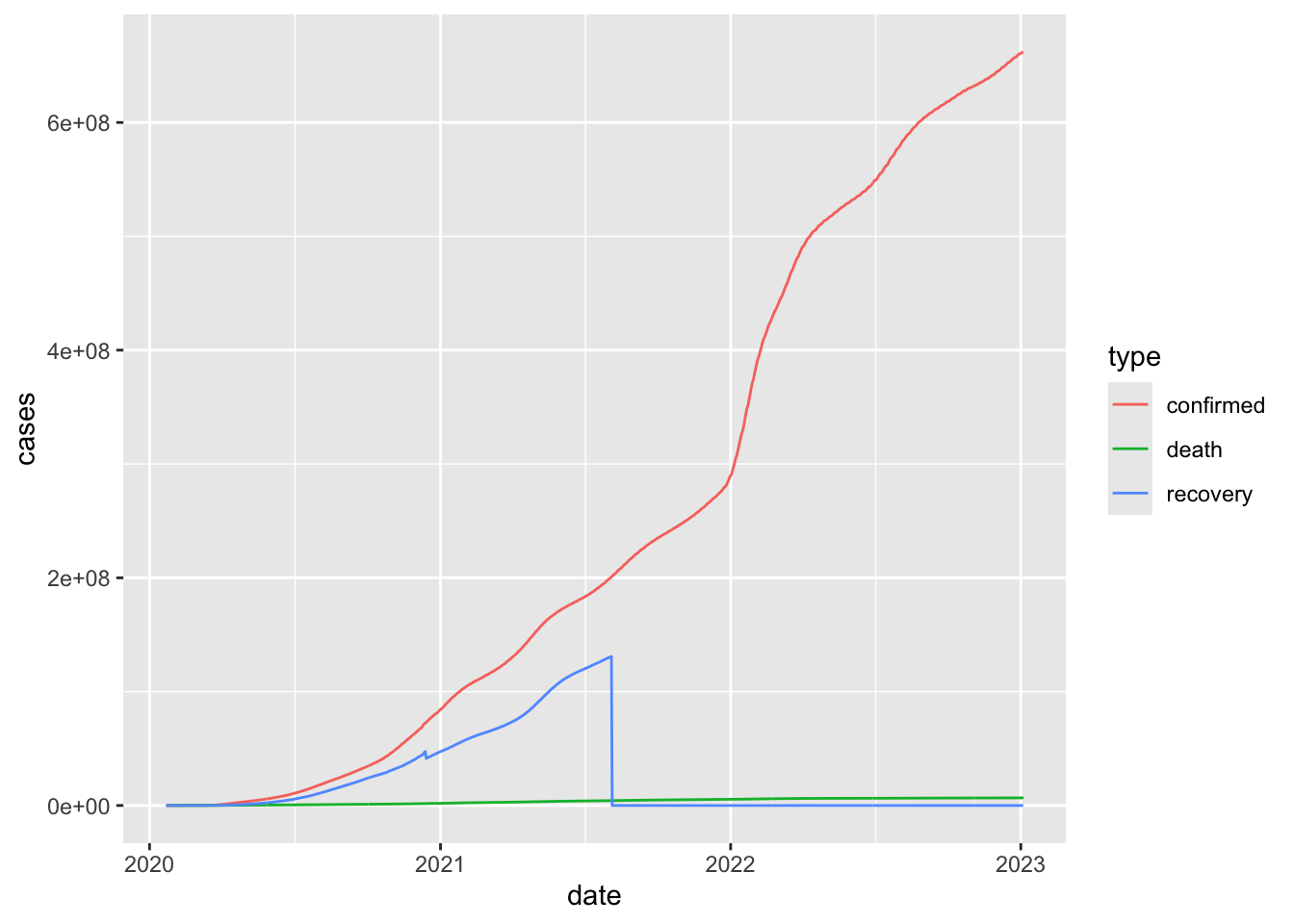## Readings

Required:

Additional resources:

## Announcements

• No class on Wednesday
• We’re half-way through the course! We will send around a mid-term evaluation survey later this week and would really appreciate your feedback. All responses will be completely anonymous

## Today’s learning objectives

The goal for today’s class is to return to ggplot to get more practice with using this package for data visualization, cover a few additional aspects of its functionality, and integrate the dplyr data wrangling skills we learned last week to further customize our plots.

By the end of this class, you should be able to:

• Use different geometric objects and aesthetics to explore various plot types in ggplot
• Chain together steps for data wrangling (dplyr) and plotting (ggplot)

If we have time, we will wrap up with a brief discussion of good coding practices.

Acknowledgements: Today’s lecture borrows from several excellent resources including the R for Excel users course by Julia Stewart Lowndes and Allison Horst and Chapter 3 of Grolemund and Wickham’s R for Data Science.

## Recap on the Grammar of Graphics implemented in ggplot

This overview is borrowed from the STAT545 course at UBCYou can think of the grammar of graphics as a systematic approach for describing the components of a graph. It has seven components (the ones in bold are required to be specifed explicitly in ggplot2):

• Data
• Exactly as it sounds: the data that you’re feeding into a plot.
• Aesthetic mappings
• This is a specification of how you will connect variables (columns) from your data to a visual dimension. These visual dimensions are called “aesthetics”, and can be (for example) horizontal positioning, vertical positioning, size, colour, shape, etc.
• Geometric objects
• This is a specification of what object will actually be drawn on the plot. This could be a point, a line, a bar, etc.
• Scales
• This is a specification of how a variable is mapped to its aesthetic. Will it be mapped linearly? On a log scale? Something else?
• Statistical transformations
• This is a specification of whether and how the data are combined/transformed before being plotted. For example, in a bar chart, data are transformed into their frequencies; in a box-plot, data are transformed to a five-number summary.
• Coordinate system
• This is a specification of how the position aesthetics (x and y) are depicted on the plot. For example, rectangular/cartesian, or polar coordinates.
• Facet
• This is a specification of data variables that partition the data into smaller “sub plots”, or panels.

These components are like parameters of statistical graphics, defining the “space” of statistical graphics. In theory, there is a one-to-one mapping between a plot and its grammar components, making this a useful way to specify graphics.

## Getting started

We’ll return to exploring the Coronavirus dataset. I’ll try to focus on typical beginner’s errors along the way so we can get used to trouble-shooting together.

Before we start, please open the R Project associated with your class GitHub repository. Then create a new RMarkdown file, select github_document output format, and save it to your lecture note folder. Copy today’s template for in-class exercises into your RMarkdown document and save it. You can follow along in this worksheet as we go through the lecture.

First, let’s load our packages and read in our Coronavirus dataset

suppressPackageStartupMessages(library(tidyverse))
suppressPackageStartupMessages(library(knitr))

coronavirus <- read_csv('https://raw.githubusercontent.com/RamiKrispin/coronavirus/master/csv/coronavirus.csv', col_types = cols(province = col_character()))

# Has it been updated? Check the latest date?
max(coronavirus$date) ##  "2021-04-10" ## Combining dplyr and ggplot Let’s start with summarizing the total number of cases by type as of the most recent day in the dataset: 2021-04-10. Take a minute to try this for yourself, then you can look at our approach. click to see our approach total_cases <- coronavirus %>% group_by(type) %>% summarize(cases = sum(cases)) kable(total_cases) # kable() just provides a nice output for the table type cases confirmed 135355885 death 2927807 recovered 83189205 Now, let’s plot the history of the daily counts of new confirmed cases worldwide # We first have to summarize the data, then plot those summary statistics # Note that we can pipe dplyr output directly into ggplot coronavirus %>% filter(type == "confirmed") %>% group_by(date) %>% summarize(total_cases = sum(cases)) %>% ggplot(mapping = aes(x = date, y = total_cases)) + geom_line()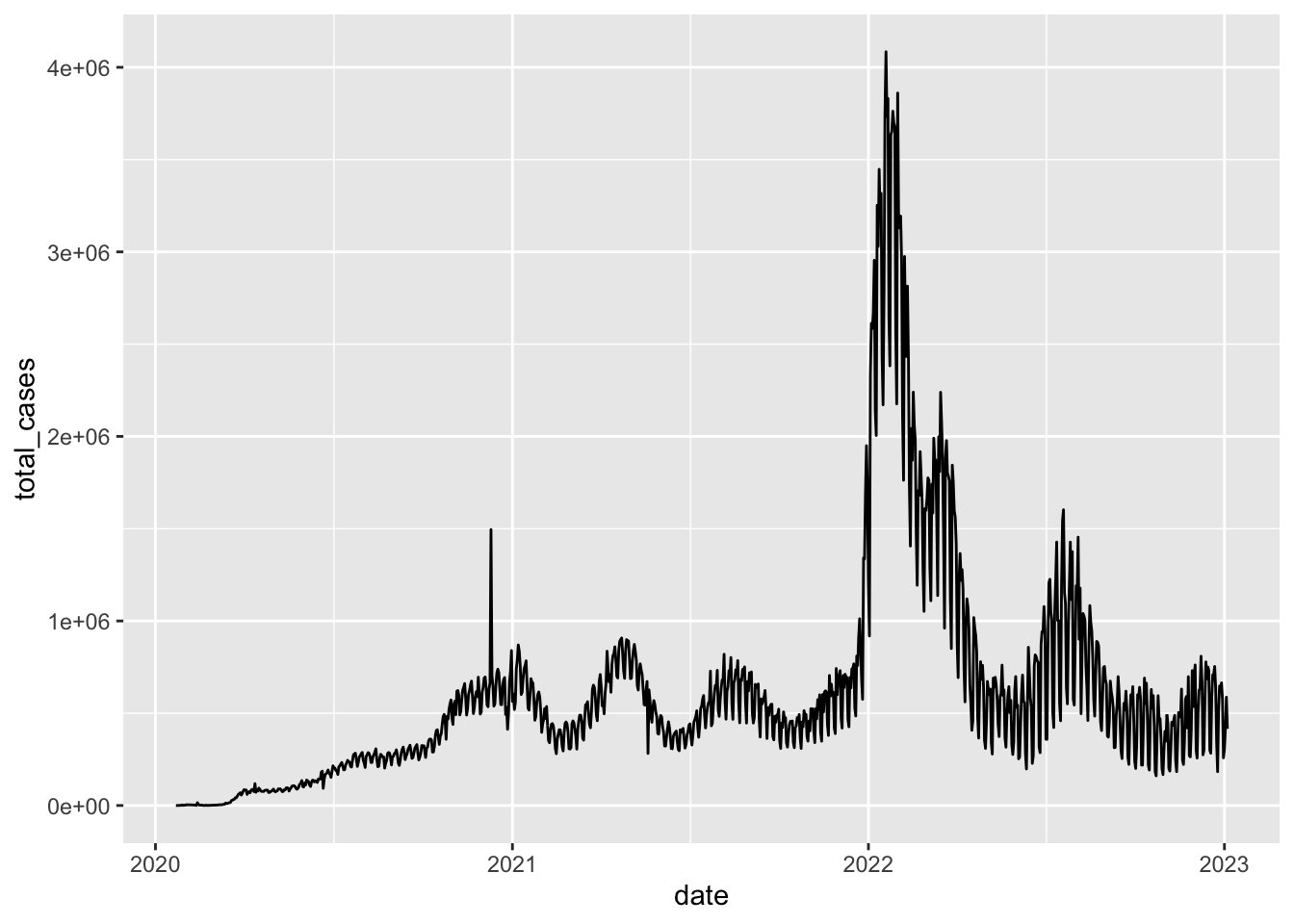If we want to play around with different geoms, we can store dplyr data processing steps and initiation of the ggplot as object gg_base so that we don’t need to retype it each time gg_base <- coronavirus %>% filter(type == "confirmed") %>% group_by(date) %>% summarize(total_cases = sum(cases)) %>% ggplot(mapping = aes(x = date, y = total_cases))  Then when we want to draw the plot, we can just call that object and specify the geom gg_base + geom_line()Try these gg_base + geom_point()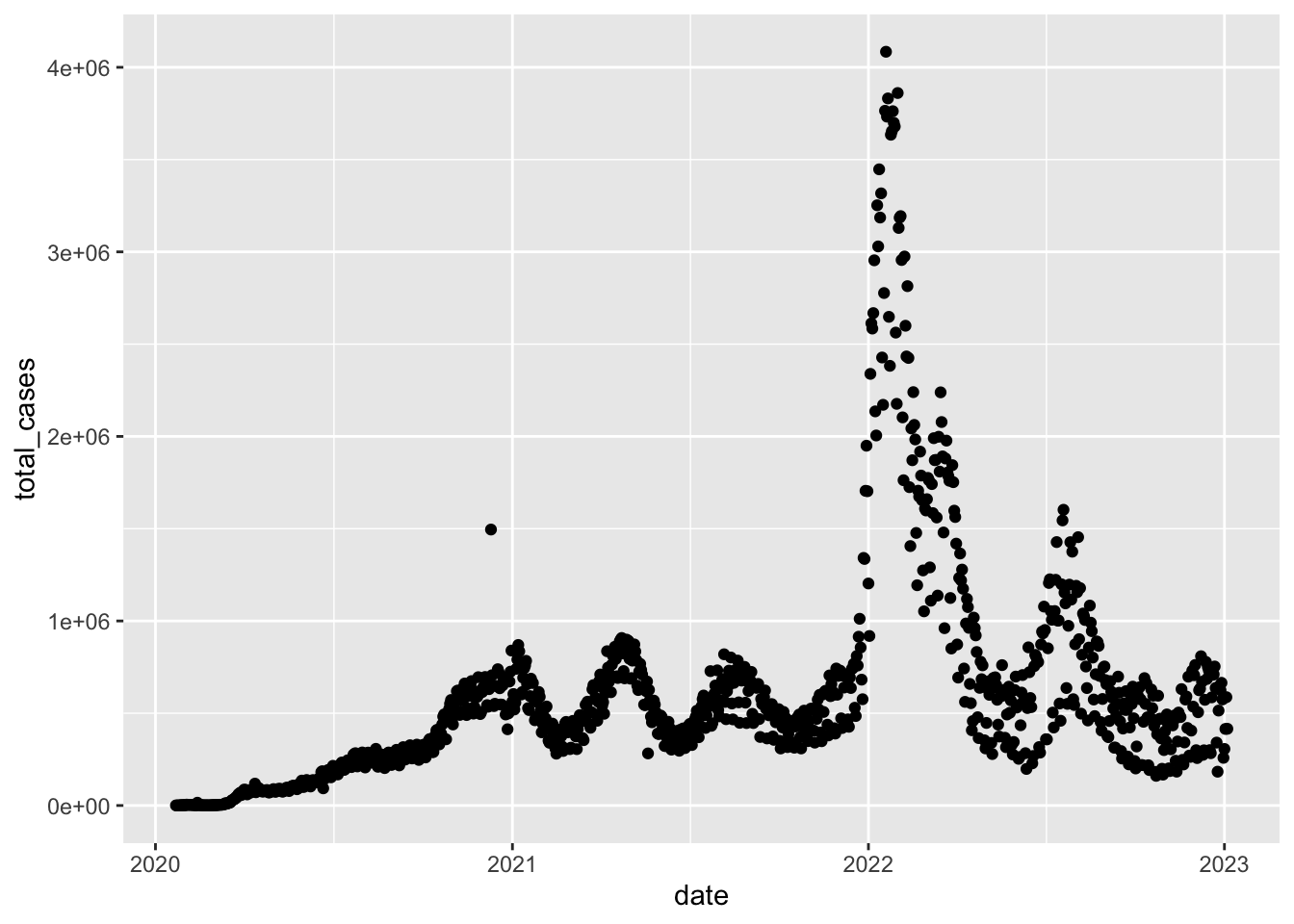gg_base + geom_col(color="red")gg_base + geom_area()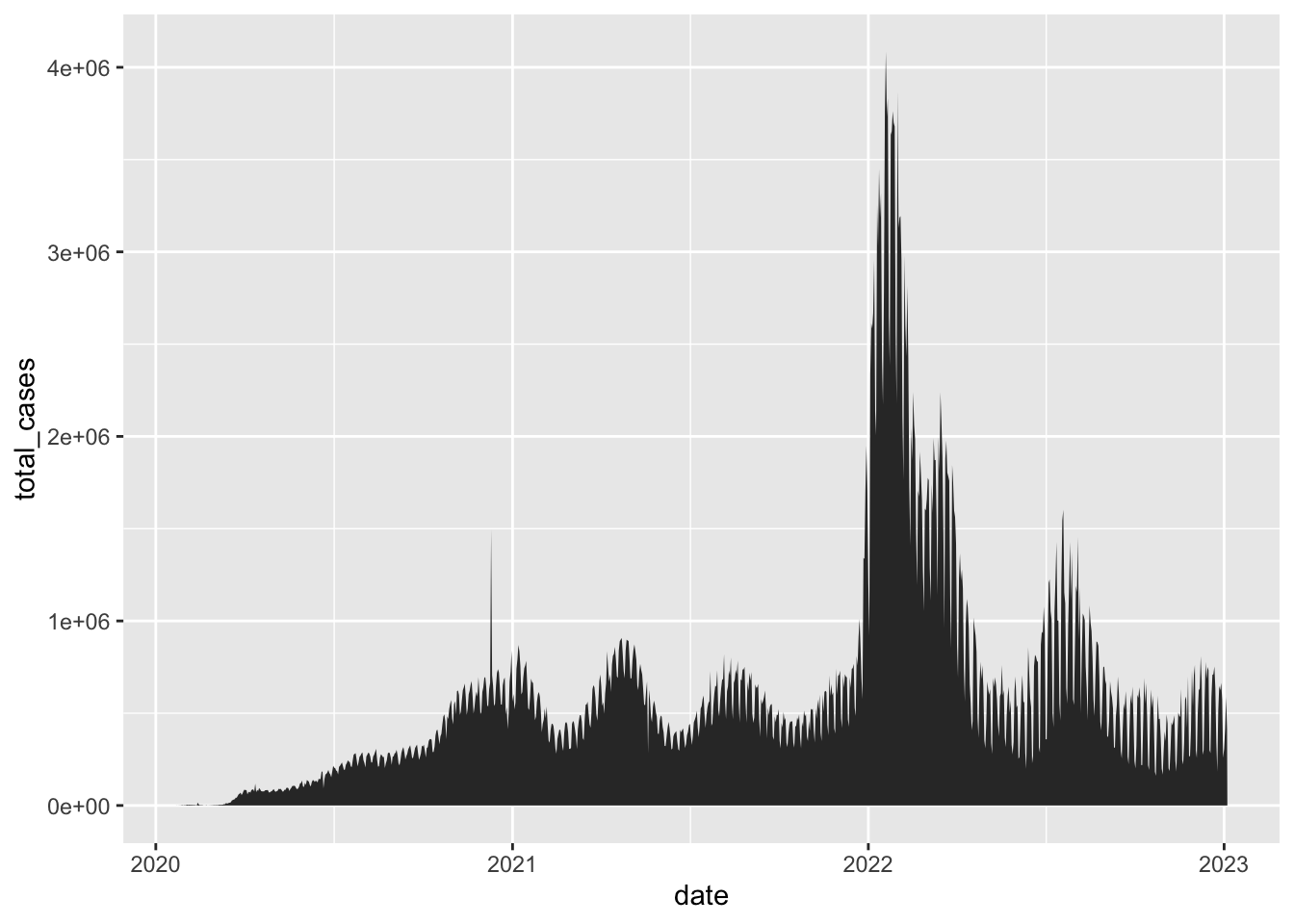## Customizing plots First, a quick reminder on how we can customize some aesthetics (e.g. colors, styles, axis labels, etc.) of our graphs based on non-variable values. We can change the aesthetics of elements in a ggplot graph by adding arguments within the layer where that element is created. Some common arguments we’ll use first are: • color =: update point or line colors • fill =: update fill color for objects with areas • linetype =: update the line type (dashed, long dash, etc.) • shape =: update the point style • size =: update the element size (e.g. of points or line thickness) • alpha =: update element opacity (1 = opaque, 0 = transparent) Building on our first line graph, let’s update the line color to “purple” and make the line type “dashed”: gg_base + geom_line( color = "purple", linetype = "dashed" )How do we know which color names ggplot will recognize? If you google “R colors ggplot2” you’ll find a lot of good resources. Here’s one: SAPE ggplot2 colors quick reference guide Now let’s update the point, style and size of points on our previous scatterplot graph using color =, size =, alpha =, and shape = (see ?pch for the different point styles, which can be further customized). gg_base + geom_point(color = "purple", shape = 17, size = 4, alpha = 0.5)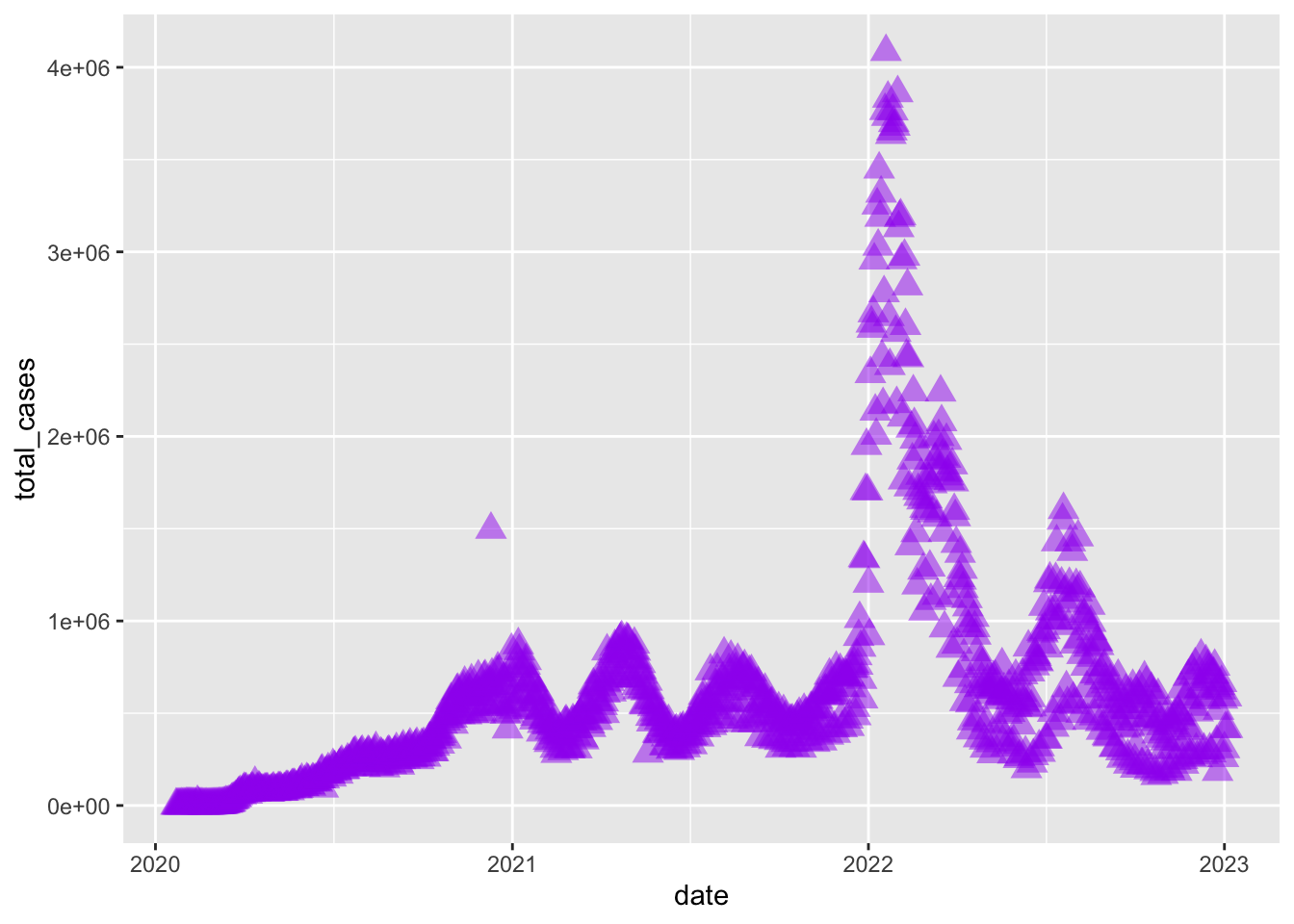## Mapping variables onto aesthetics In the examples above, we have customized aesthetics based on constants that we input as arguments (e.g., the color / style / size isn’t changing based on a variable characteristic or value). Often, however, we do want the aesthetics of a graph to depend on a variable. To do that (as we’ve discussed earlier), we’ll map variables onto graph aesthetics, meaning we’ll change how an element on the graph looks based on a variable characteristic (usually, character or value). When we want to customize a graph element based on a variable’s characteristic or value, add the argument within aes() in the appropriate geom_*() layer. In short, if updating aesthetics based on a variable, make sure to put that argument inside of aes(). Example: Create a ggplot scatterplot graph where the size and color of the points change based on the number of cases, and make all points the same level of opacity (alpha = 0.5). Notice the aes() around the size = and color = arguments. Note: this is just for illustration of the functionality only - we are showing the same information in redundant ways, which is typically not helpful or necessary. Avoid excessive / overcomplicated aesthetic mapping in data visualization. gg_base + geom_point( aes(size = total_cases, color = total_cases), alpha = 0.5 )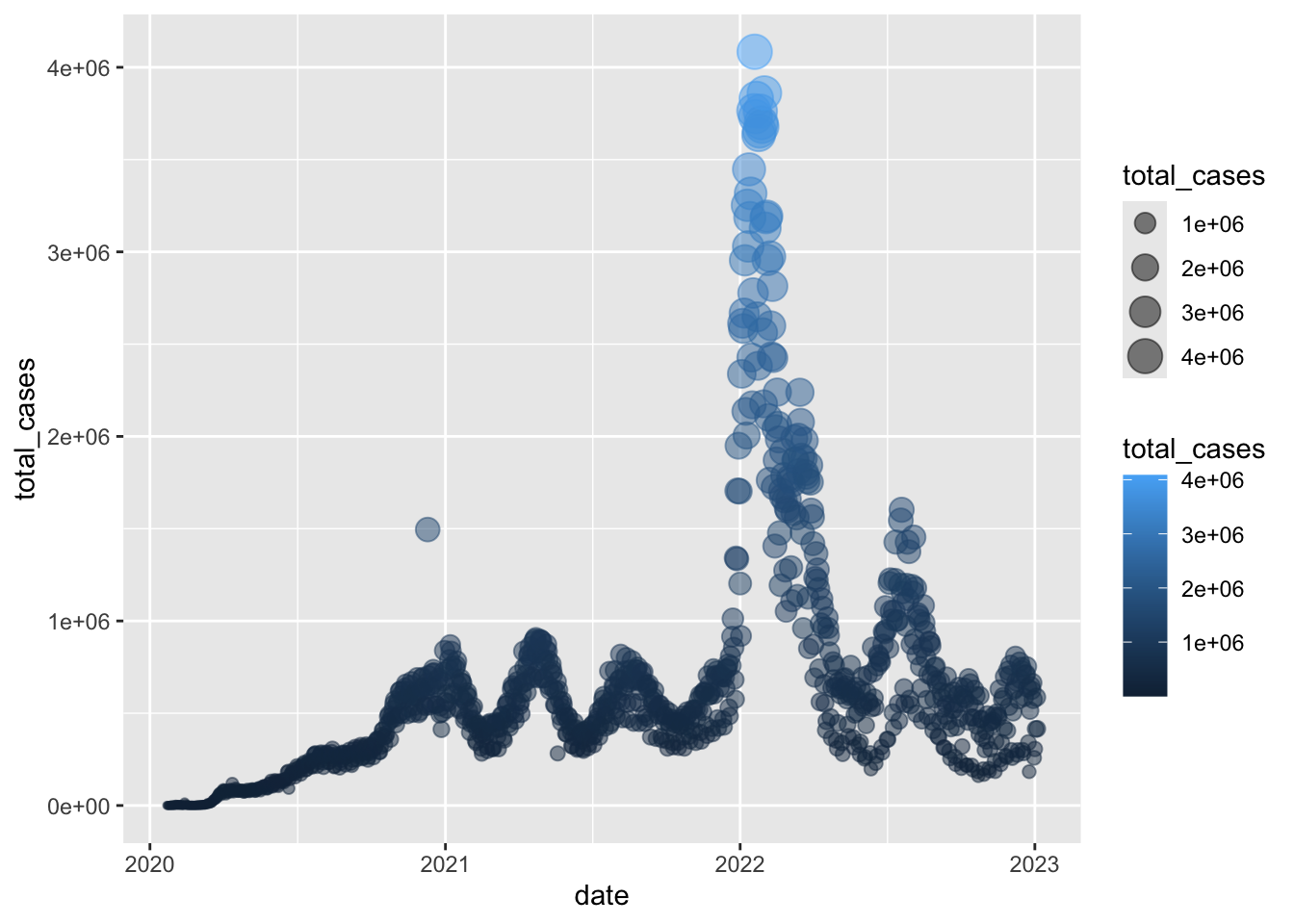In the example above, notice that the two arguments that do depend on variables are within aes(), but since alpha = 0.5 doesn’t depend on a variable then it is outside the aes() but still within the geom_point() layer. When we map variables to aesthetics, ggplot2 will automatically assign a unique level of the aesthetic (here a unique color) to each unique value of the variable, a process known as scaling. ggplot2 will also add a legend that explains which levels correspond to which values. ## ggplot2 complete themes While every element of a ggplot graph is manually customizable, there are also built-in themes (theme_*()) that you can add to your ggplot code to make some major headway before making smaller tweaks manually. We talked about this briefly a few classes ago, but let’s explore a little further. Here are a few to try today (but also notice all the options that appear as we start typing theme_ into our ggplot graph code!): • theme_light() • theme_minimal() • theme_bw() Also, check out more examples by scrolling down here. Pick one that you like and update your previous plot. Here, let’s update our previous graph with theme_minimal(): gg_base + geom_point( aes(size = total_cases, color = total_cases), alpha = 0.5 ) + theme_minimal()You can play around with other themes - see an overview and instructions for how to customize themes here ## Updating axis labels and titles Use labs() to update axis labels, and add a title and/or subtitle to your ggplot graph. gg_base + geom_line(linetype = "dotted") + theme_bw() + labs( x = "Date", y = "Total confirmed cases", title = "Daily counts of new Coronavirus cases recorded", subtitle = "Global sums" )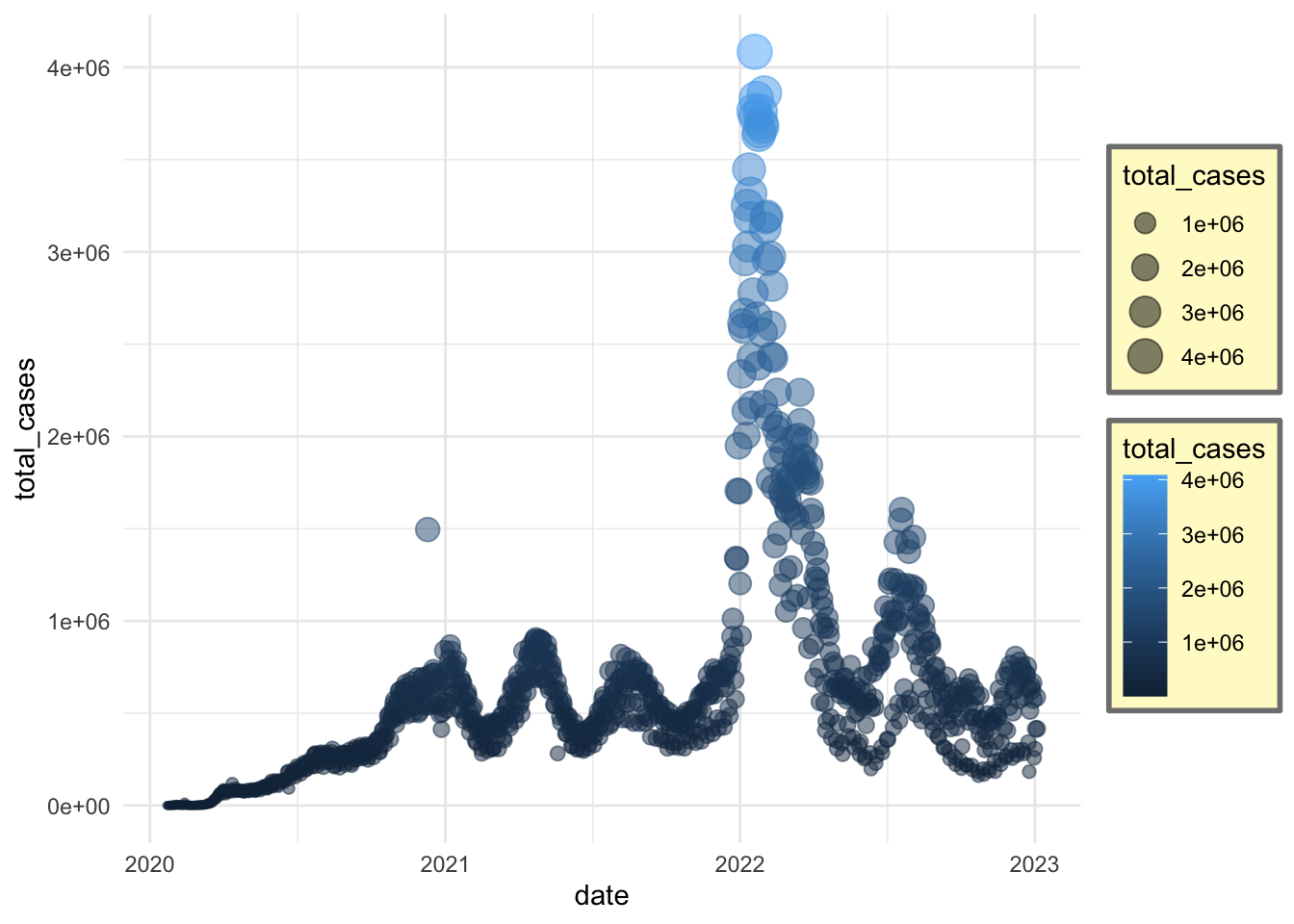Note: If you want to update the formatting of axis values (for example, to convert to comma format instead of scientific format above), you can use the scales package options and add + scale_y_continuous(labels = scales::comma) (see more from the R Cookbook). ## Now, let’s split the case counts out by country ### Your turn Take a minute to think about how you would generate a plot with a separate line showing the daily reports of new confirmed cases in each country. Here is some code we might try. Why does that not work? coronavirus %>% filter(type == "confirmed") %>% group_by(date) %>% summarize(total_cases = sum(cases)) %>% ggplot() + geom_line(mapping = aes(x = date, y = total_cases, color = country)) # We have summarized out the country details (only one total count per day) We’ll have to group by both country and date. But why does this not work? coronavirus %>% filter(type == "confirmed") %>% group_by(country, date) %>% summarize(total_cases = sum(cases)) %>% ggplot(mapping = aes(x = date, y = total_cases)) + geom_line() # Even though we have grouped the dataframe by country, that dplyr grouping does not get carried into ggplot Now let’s make ggplot group the data too by mapping country onto an aesthetic coronavirus %>% filter(type == "confirmed") %>% group_by(country, date) %>% summarize(total_cases = sum(cases)) %>% ggplot(mapping = aes(x = date, y = total_cases, color = country)) + geom_line() ## summarise() has grouped output by 'country'. You can override using the .groups argument.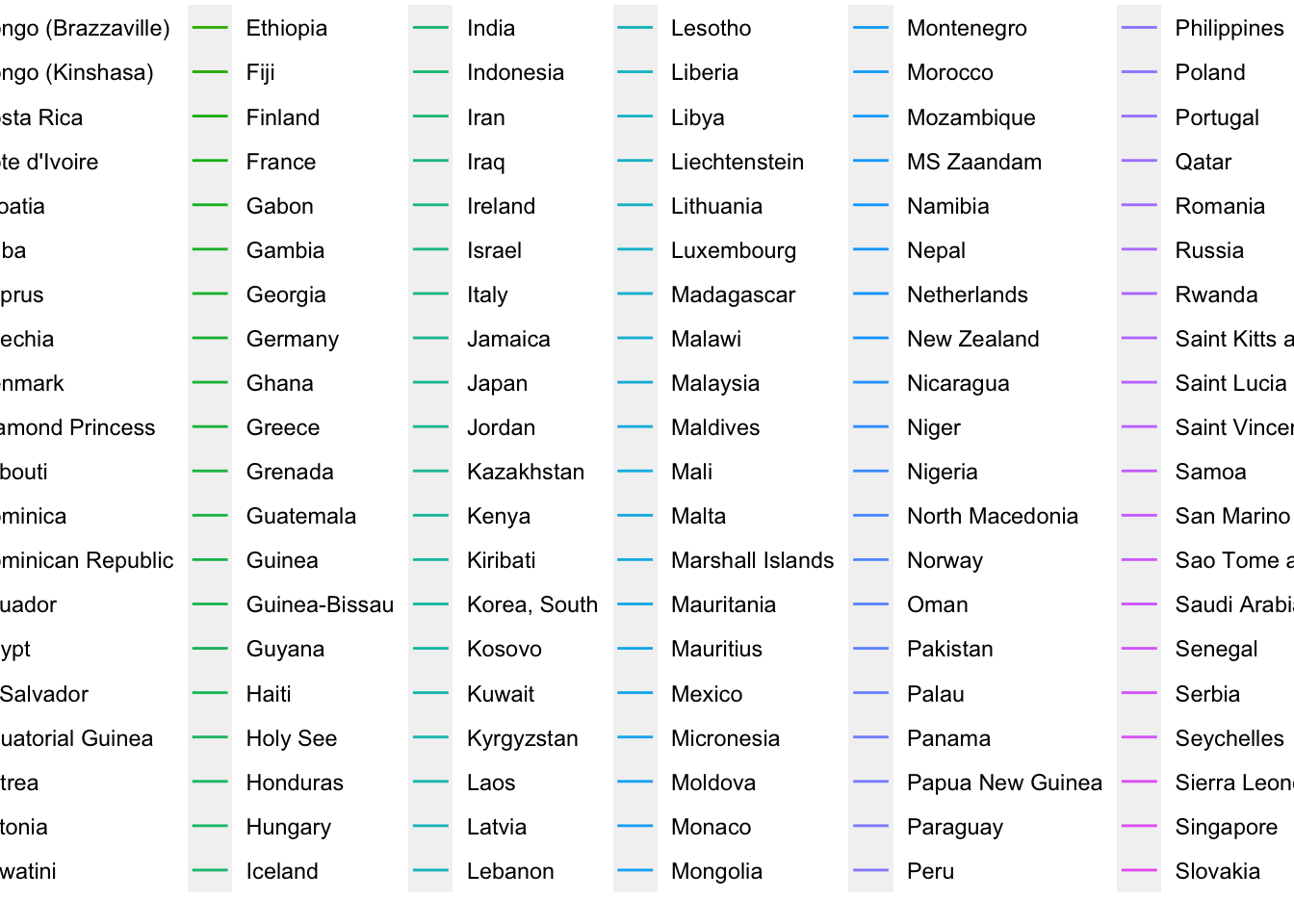It looks like this is doing what we want, but it does not display well. There are too many countries! We could play around with the layout parameters to be able to see this plot. But let’s instead subset to only show the 10 countries with the highest total counts of confirmed cases. top10_countries <- coronavirus %>% filter(type == "confirmed") %>% group_by(country) %>% summarize(total_cases = sum(cases)) %>% arrange(-total_cases) %>% head(10) %>% .$country

Now let’s try to plot the daily counts of new cases just for those countries

coronavirus %>%
filter(type == "confirmed", country %in% top10_countries) %>%
group_by(country, date) %>%
summarize(total_cases = sum(cases)) %>%  # Need this summarize because some countries have data broken down by Province.State
ggplot(mapping = aes(x = date, y = total_cases, color = country)) +
geom_line()
## summarise() has grouped output by 'country'. You can override using the .groups argument.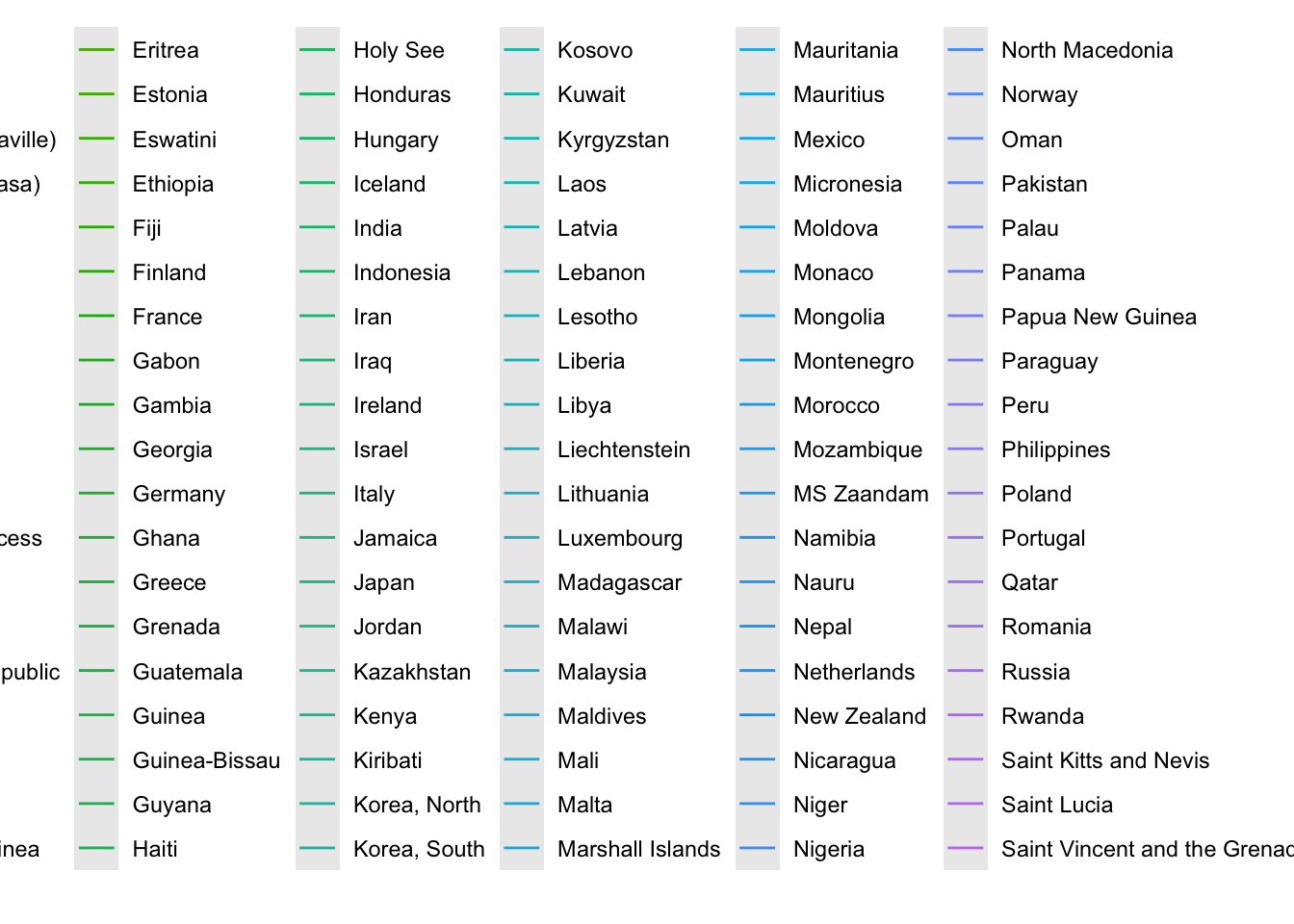Much better!

We can also make a separate panel for each country

coronavirus %>%
filter(type == "confirmed", country %in% top10_countries) %>%
group_by(country, date) %>%
summarize(total_cases = sum(cases)) %>%
ggplot(mapping = aes(x = date, y = total_cases)) +
geom_line() +
facet_wrap(~country)
## summarise() has grouped output by 'country'. You can override using the .groups argument.Now let’s plot the cumulative sum of cases for each of those countries instead

coronavirus %>%
filter(type == "confirmed", country %in% top10_countries) %>%
group_by(country) %>%
arrange(date) %>%
mutate(cum_count = cumsum(cases)) %>%
ggplot(mapping = aes(x = date, y = cum_count, color = country)) +
geom_line()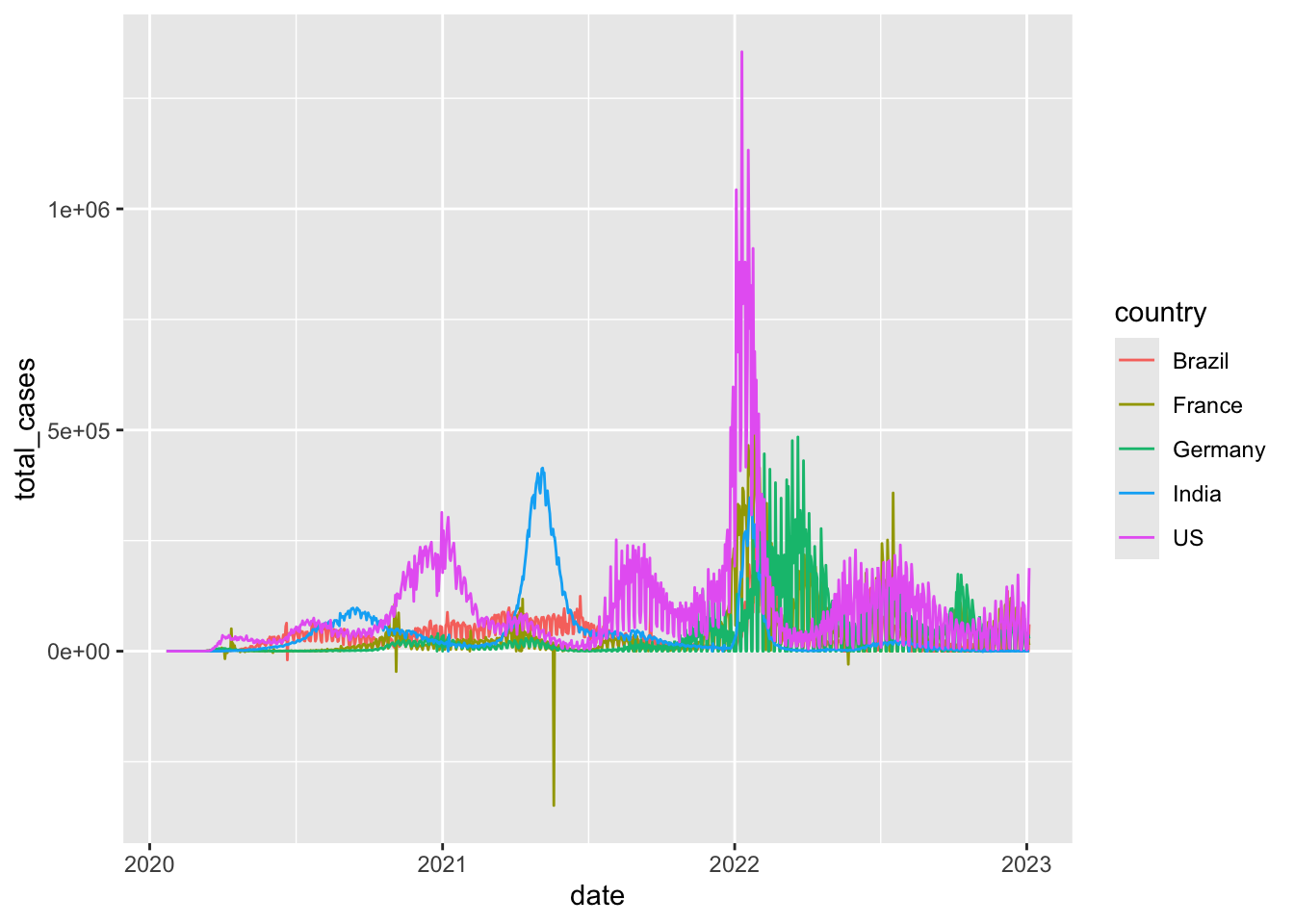## Bar charts

Another common plot type are bar charts. There are two types of bar charts in ggplot: geom_bar() and geom_col(). geom_bar() makes the height of the bar proportional to the number of cases in each group (or if the weight aesthetic is supplied, the sum of the weights). If you want the heights of the bars to represent values in the data, use geom_col() instead.

Since our dataset reports counts of cases, let’s first start with geom_col() (we have already used it once today).

coronavirus %>%
group_by(date) %>%
summarize(cases = sum(cases)) %>%
ggplot() +
geom_col(aes(x = date, y = cases), color = "black")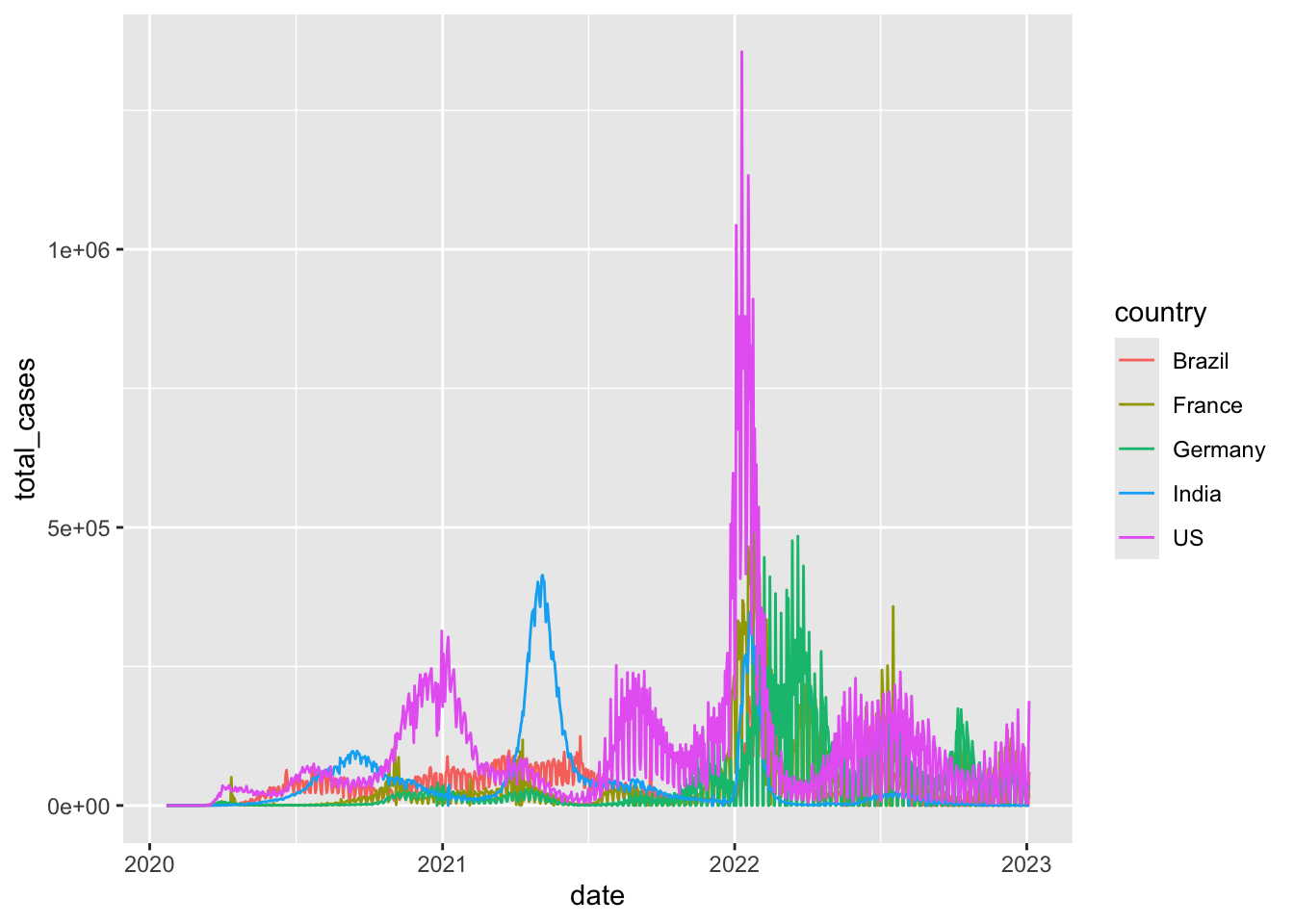If we want a stacked barplot separating out the different types of cases, we can use the fill aesthetic

coronavirus %>%
group_by(date, type) %>%
summarize(cases = sum(cases)) %>%
ggplot() +
geom_col(aes(x = date, y = cases, fill = type), size=0.1)
## summarise() has grouped output by 'date'. You can override using the .groups argument.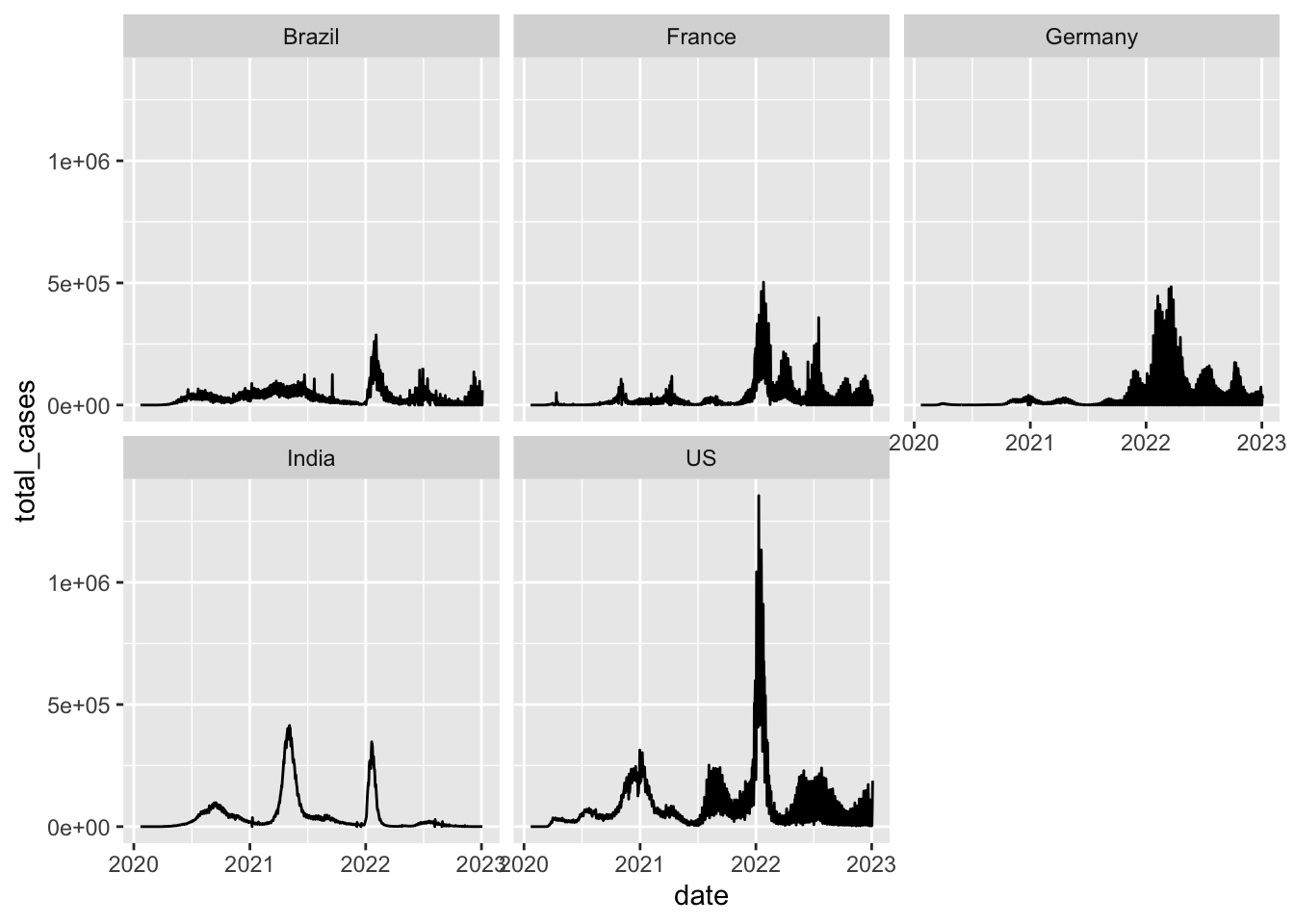If it looks like your chart is just made up of black bars, try making it bigger, or reduce the size argument (which controls the line thickness).

We may want to flip this around

coronavirus %>%
group_by(date, type) %>%
summarize(cases = sum(cases)) %>%
ggplot() +
geom_col(aes(x = date, y = cases, fill = type), size=0.1) +
coord_flip()
## summarise() has grouped output by 'date'. You can override using the .groups argument.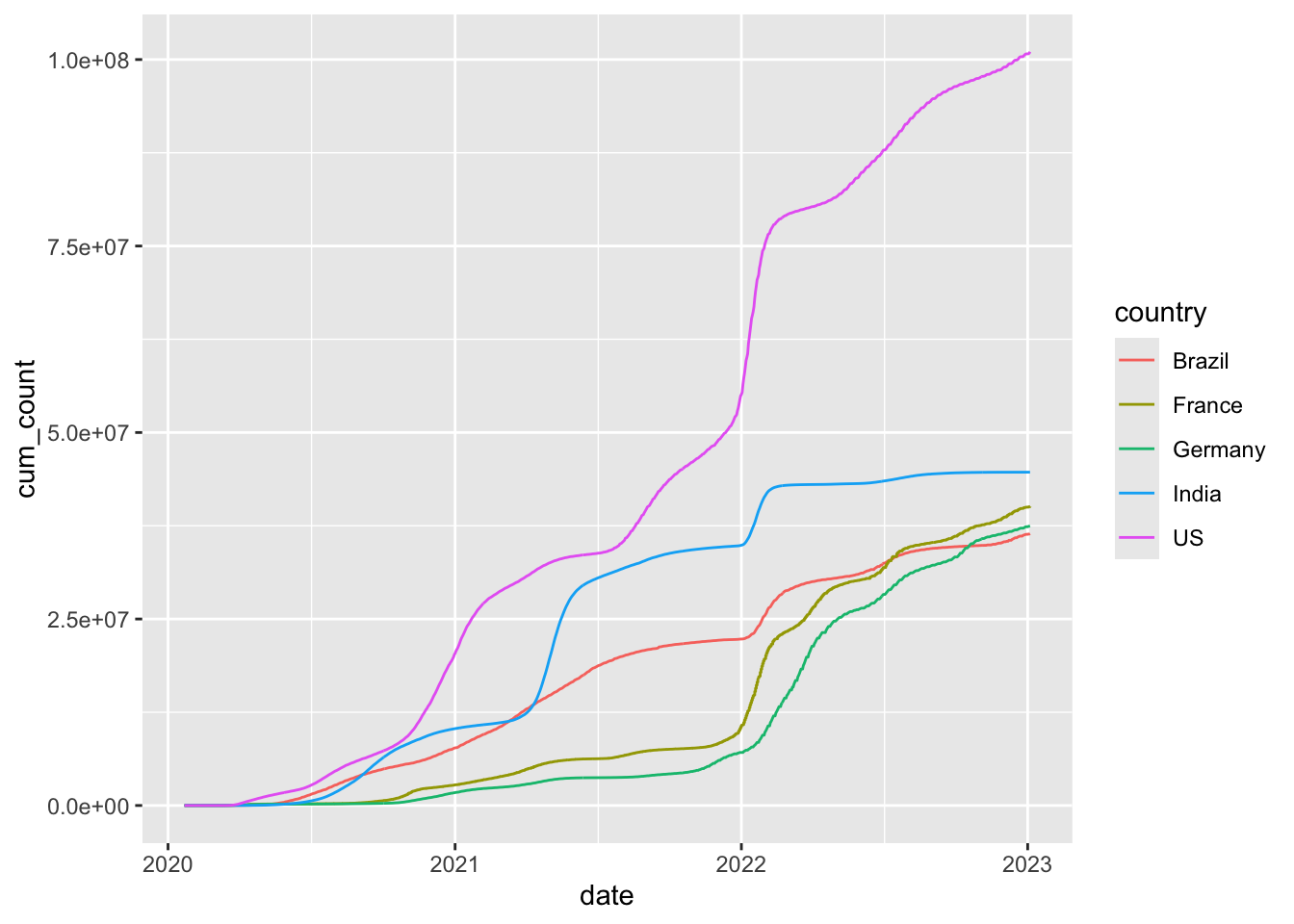This is useful because it puts the proportions in relation to the total daily counts. But it can be hard to compare proportions. We can make all bars the same height with ‘position adjustment’

coronavirus %>%
group_by(date, type) %>%
summarize(cases = sum(cases)) %>%
ggplot() +
geom_col(aes(x=date, y = cases, fill = type), position = "fill")
## summarise() has grouped output by 'date'. You can override using the .groups argument.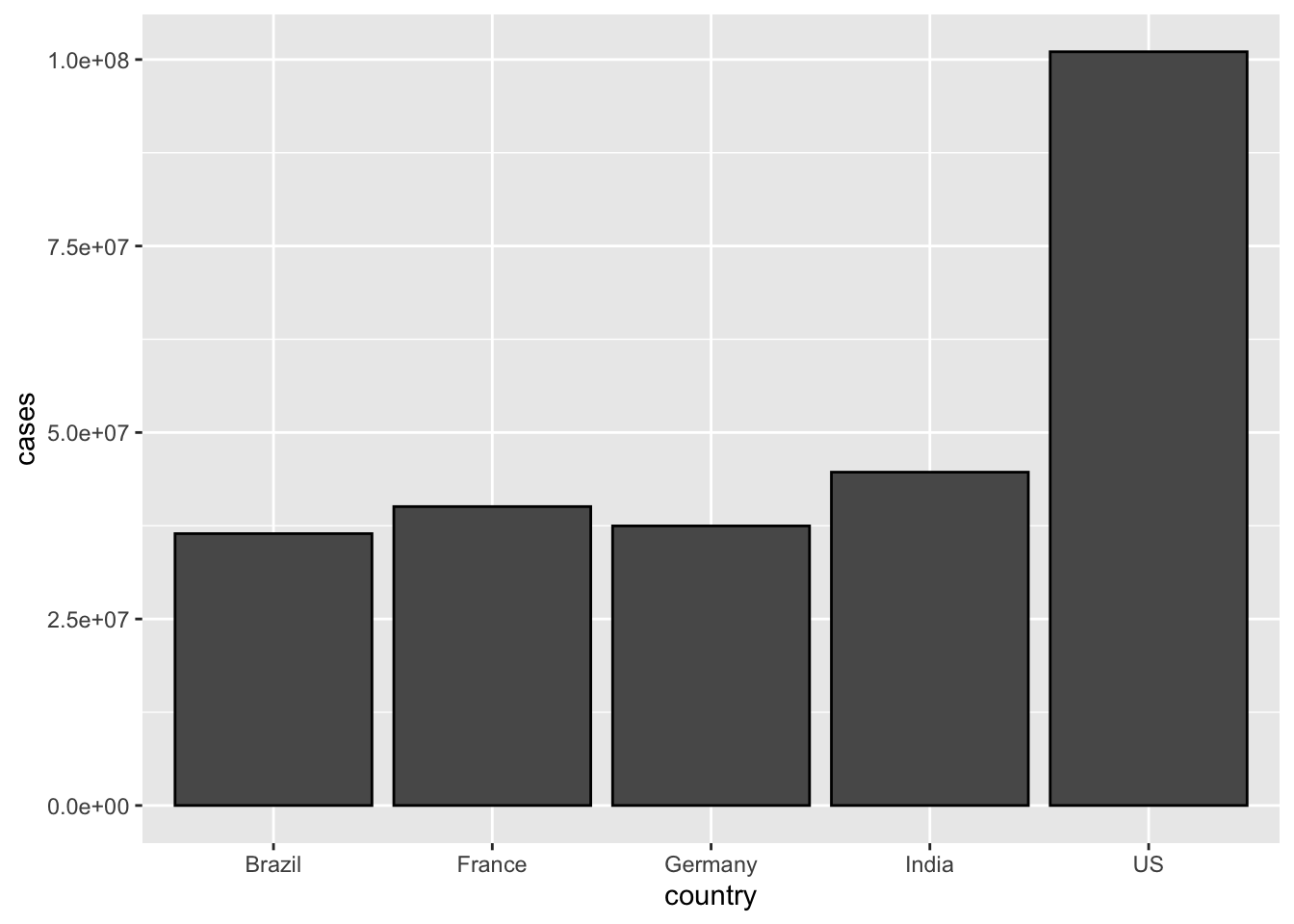Let’s check if we have negative cases.

coronavirus %>%
filter(cases < 0)
## # A tibble: 443 x 7
##    date       province                    country       lat   long type    cases
##    <date>     <chr>                       <chr>       <dbl>  <dbl> <chr>   <dbl>
##  1 2020-01-31 Queensland                  Australia  -27.5   153.  confir…    -1
##  2 2020-02-02 Queensland                  Australia  -27.5   153.  confir…    -1
##  3 2020-03-06 Northern Territory          Australia  -12.5   131.  confir…    -1
##  4 2020-03-09 Saint Barthelemy            France      17.9   -62.8 confir…    -2
##  5 2020-03-11 NA                          Israel      31.0    34.9 confir…   -28
##  6 2020-03-18 Guizhou                     China       26.8   107.  confir…    -1
##  7 2020-03-22 Saint Helena, Ascension an… United Ki…  -7.95  -14.4 confir…    -1
##  8 2020-03-24 NA                          Guyana       4.86  -58.9 confir…   -15
##  9 2020-03-25 Alberta                     Canada      53.9  -117.  confir…    -1
## 10 2020-04-02 Turks and Caicos Islands    United Ki…  21.7   -71.8 confir…    -1
## # … with 433 more rows
# Let's remove those
coronavirus %>%
filter(cases > 0) %>%
group_by(date, type) %>%
summarize(cases = sum(cases)) %>%
ggplot() +
geom_col(aes(x = date, y = cases, fill = type), position = "fill")
## summarise() has grouped output by 'date'. You can override using the .groups argument.We can also get the bars for the different types of cases each day stacked next to each other with another position adjustment option

coronavirus %>%
filter(cases > 0) %>%
group_by(date, type) %>%
summarize(cases = sum(cases)) %>%
ggplot() +
geom_col(aes(x = date, y = cases, fill = type), position = "dodge")
## summarise() has grouped output by 'date'. You can override using the .groups argument.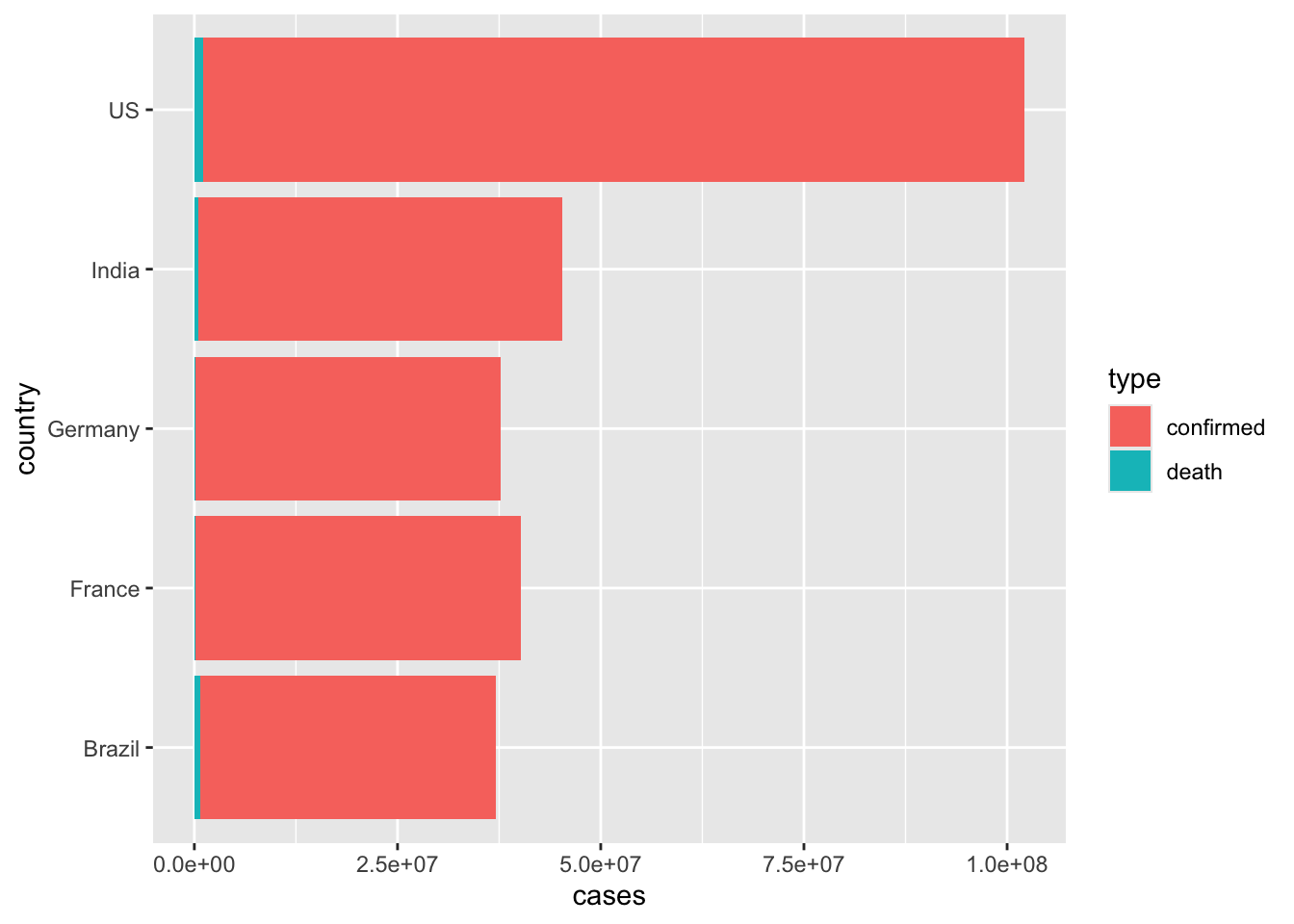Now, let’s break it down by country. Let’s again subset to only the top 10 countries with the highest counts

coronavirus %>%
filter(cases > 0, country %in% top10_countries) %>%
group_by(country, type, date) %>%
summarize(cases = sum(cases)) %>%
ggplot() +
geom_col(aes(x = date, y = cases, fill = type), position = "fill", width = 1) +
facet_wrap(~country)
## summarise() has grouped output by 'country', 'type'. You can override using the .groups argument.While looking at the proportions of case types in different areas is helpful for comparing patterns, they don’t allow for comparison of the magnitude of case counts. Let’s again scale the bars by the raw case counts.

coronavirus %>%
filter(cases > 0, country %in% top10_countries) %>%
group_by(country, type, date) %>%
summarize(cases=sum(cases)) %>%
ggplot() +
geom_col(aes(x=date, y = cases, fill = type), width = 1) +
facet_wrap(~country)
## summarise() has grouped output by 'country', 'type'. You can override using the .groups argument.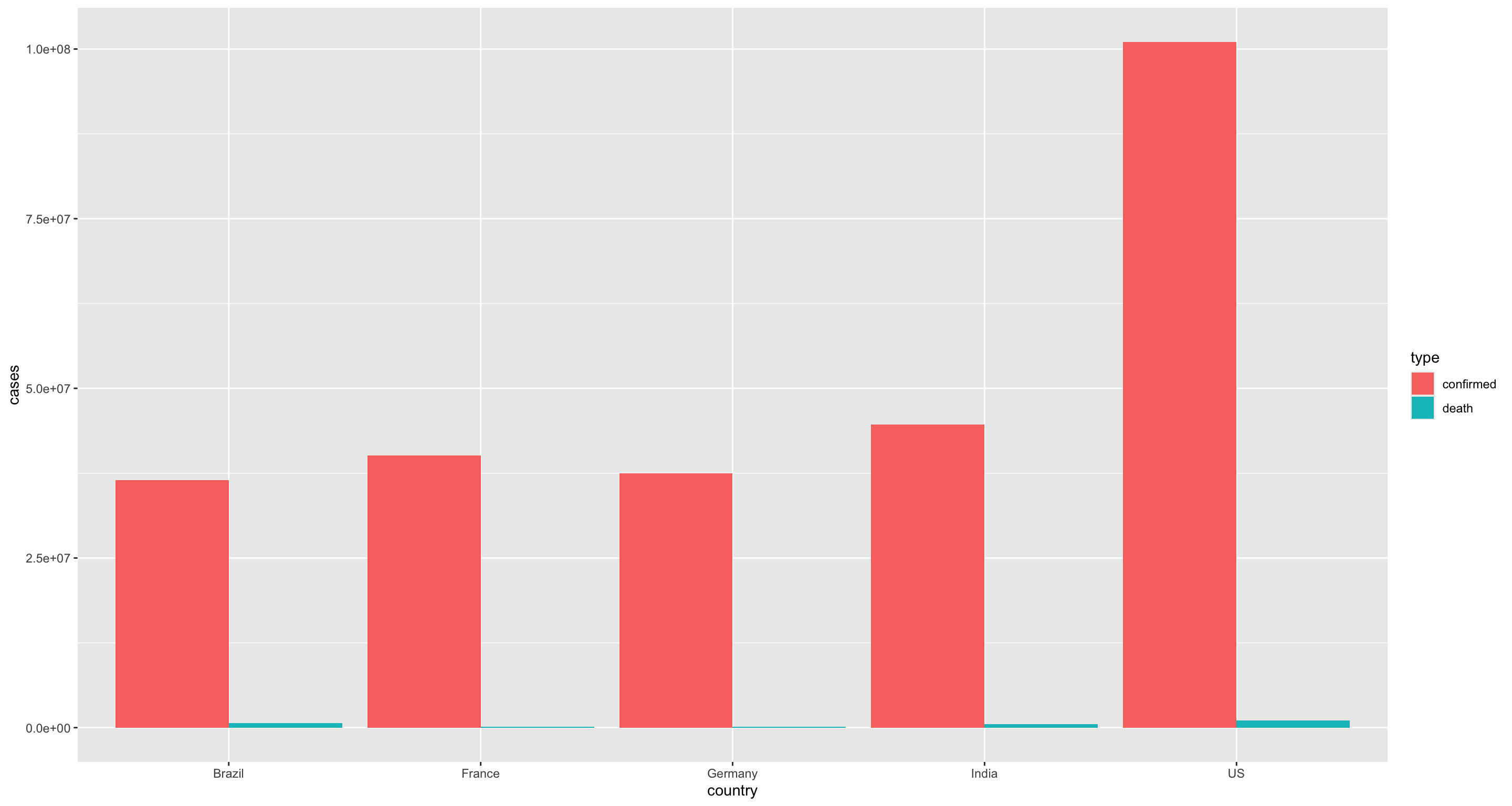Now, let’s explore a different question. For each day, let’s plot how many different countries have at least one new confirmed case. For this we will need to count rows within grouped variables, so we’ll use the geom_bar()

#How many countries have at least one confirmed case each day
coronavirus %>%
filter(type == "confirmed") %>%
group_by(country, date) %>%
summarize(total_cases = sum(cases)) %>%
filter(total_cases > 0) %>%
ggplot() +
geom_bar(mapping = aes(x = date), color="black")
## summarise() has grouped output by 'country'. You can override using the .groups argument.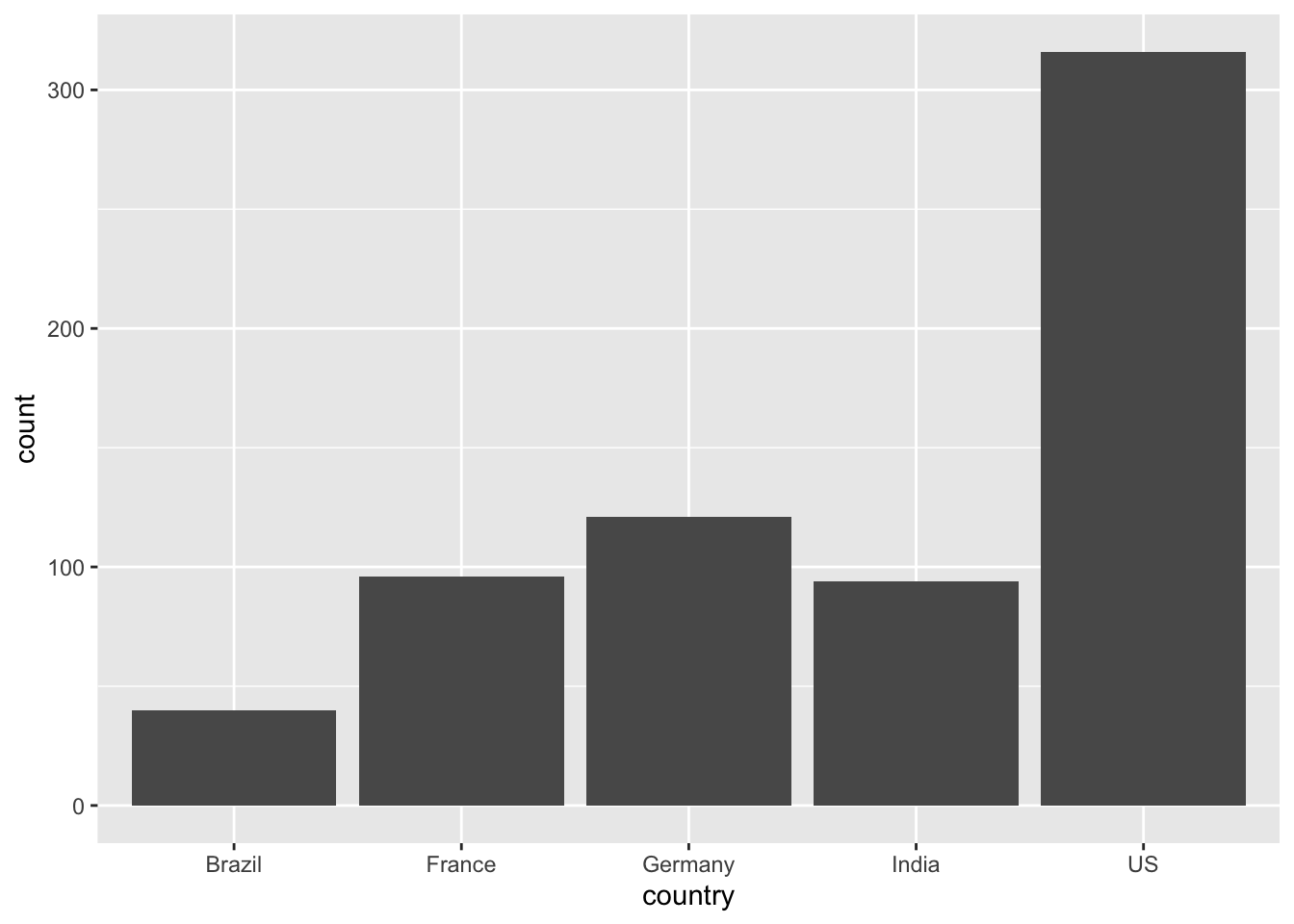## Alternatively
coronavirus %>%
filter(type == "confirmed") %>%
group_by(country, date) %>%
summarize(total_cases = sum(cases)) %>%
filter(total_cases > 0) %>%
group_by(date) %>%
summarise(count=n()) %>%
ggplot() +
geom_line(mapping = aes(x = date, y=count), color="black")
## summarise() has grouped output by 'country'. You can override using the .groups argument.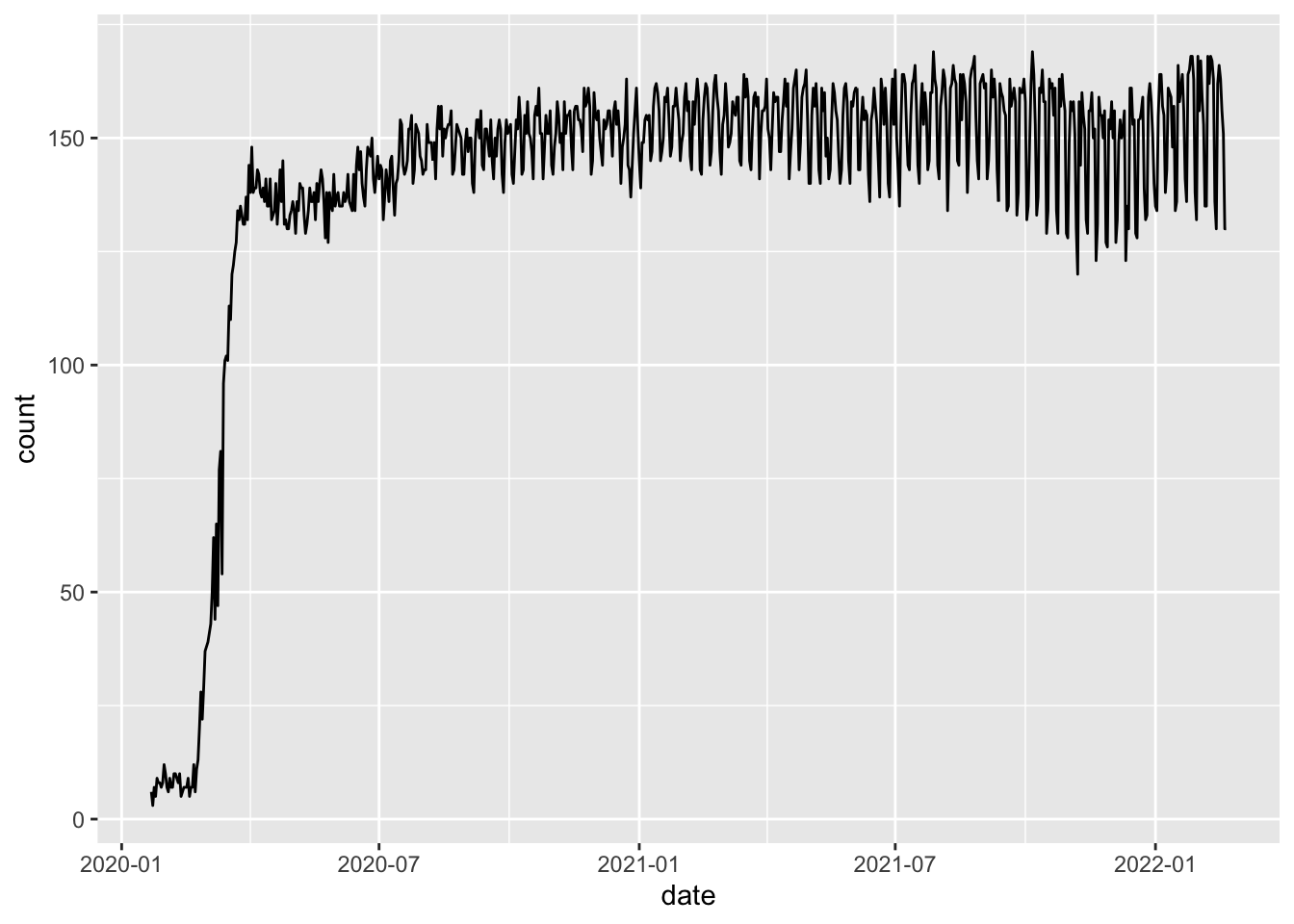## Plotting with labels

Now let’s look at the relationship between confirmed cases and deaths in each country. For this we will need our re-formatted wide data from last class.

coronavirus_ttd <- coronavirus %>%
group_by(country, type) %>%
summarize(total_cases = sum(cases)) %>%
pivot_wider(names_from = type,
values_from = total_cases) %>%
arrange(-confirmed)
## summarise() has grouped output by 'country'. You can override using the .groups argument.
coronavirus_ttd %>%
ggplot() +
geom_point(mapping = aes(x = confirmed, y = death))That’s nice, but it would be useful to know which country is represented by each dot. geom_label() is our tool for that.

coronavirus_ttd %>%
ggplot() +
geom_label(mapping = aes(x = confirmed, y = death, label = country))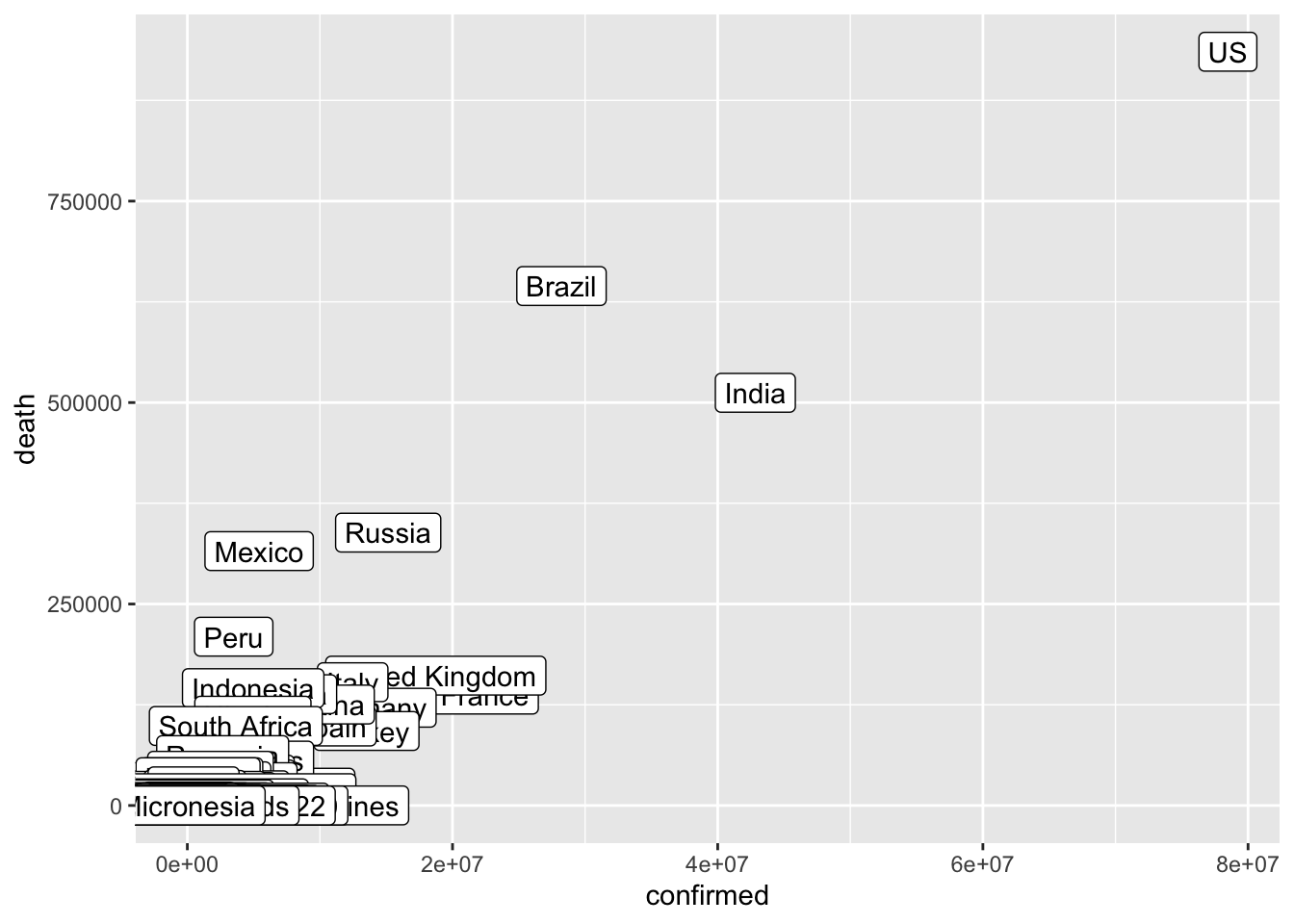Let’s do a few things to make this easier to read

We can remove countries with a small number of confirmed cases, and log transform the case counts

coronavirus_ttd %>%
filter(confirmed > 2000000) %>%
ggplot() +
geom_label(mapping = aes(x = log(confirmed), y = log(death), label = country))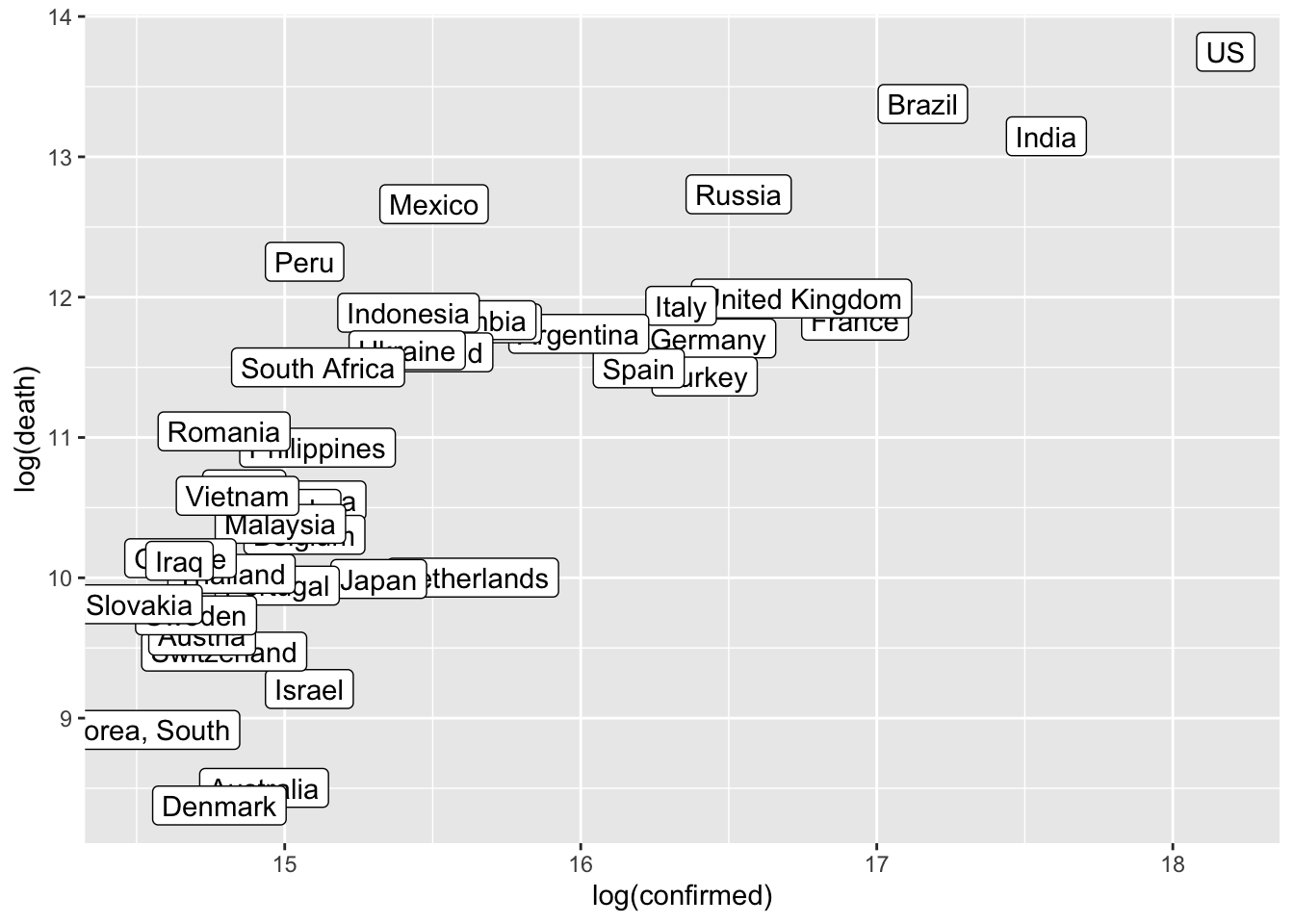Or, we can zoom in on the region with low case counts

coronavirus_ttd %>%
filter(confirmed > 0, confirmed < 20000) %>%
ggplot() +
geom_label(mapping = aes(x = confirmed, y = death, label = country))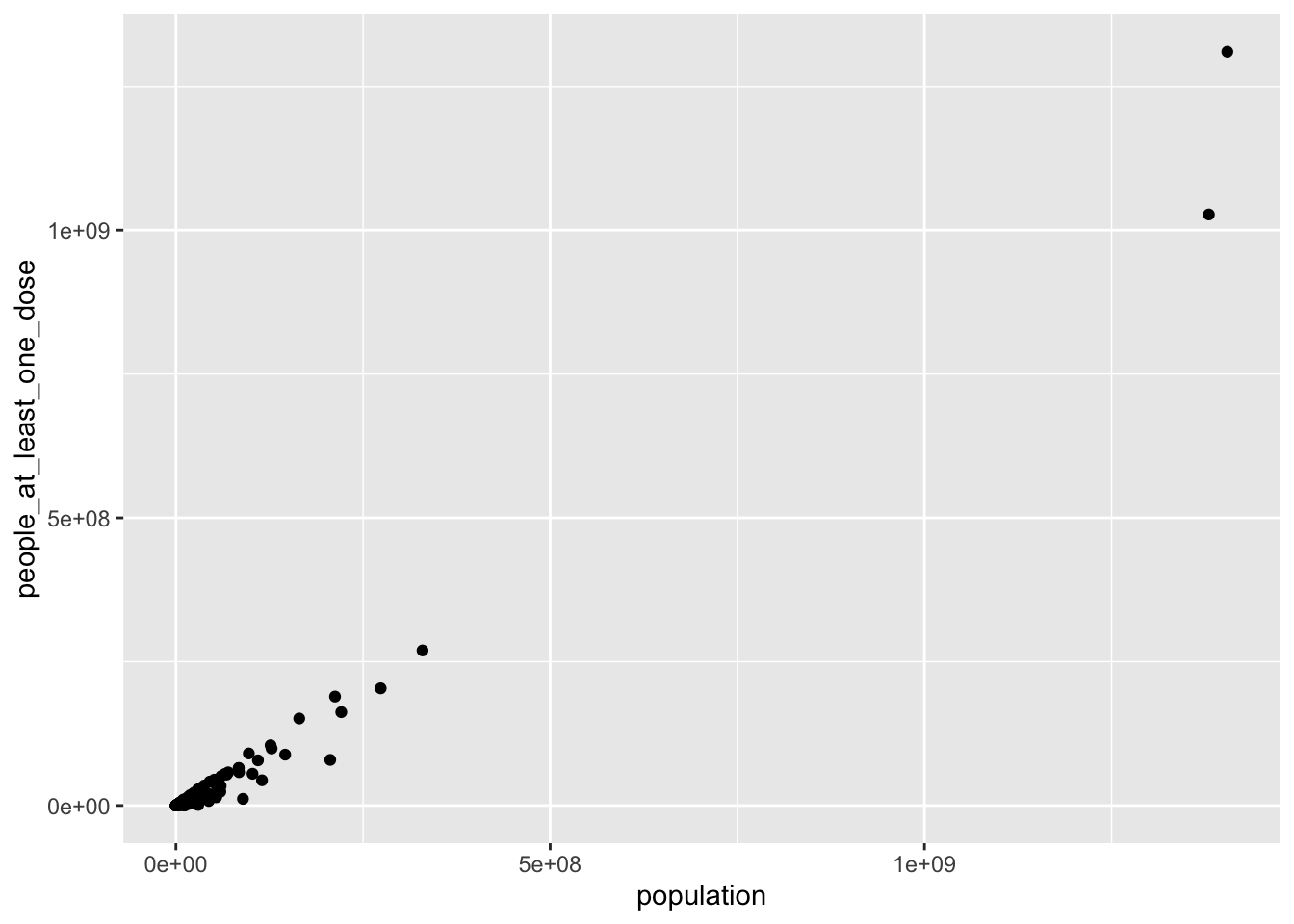### Bonus: Spatial plotting

#### Map of newly reported cases

With some additional packages, we can also plot geographical patterns on a map. We can, for example, show which countries have new counts of >5000 new confirmed cases on the most recent day from this dataset and scale the points with case counts.

library("rnaturalearth") # install.packages("rnaturalearth")
library("rnaturalearthdata") # install.packages("rnaturalearthdata")
library("rgeos") #install.packages("rgeos")
## Loading required package: sp
## rgeos version: 0.5-5, (SVN revision 640)
##  GEOS runtime version: 3.8.1-CAPI-1.13.3
##  Linking to sp version: 1.4-2
##  Polygon checking: TRUE
world <- ne_countries(scale = "medium", returnclass = "sf")
filter(coronavirus, date == max(coronavirus\$date), type == "confirmed", cases > 5000) %>%
ggplot() +
geom_sf(data = world) +
geom_point(aes(x=long, y=lat, size=cases), color="red", fill="red", alpha=0.5, shape=21)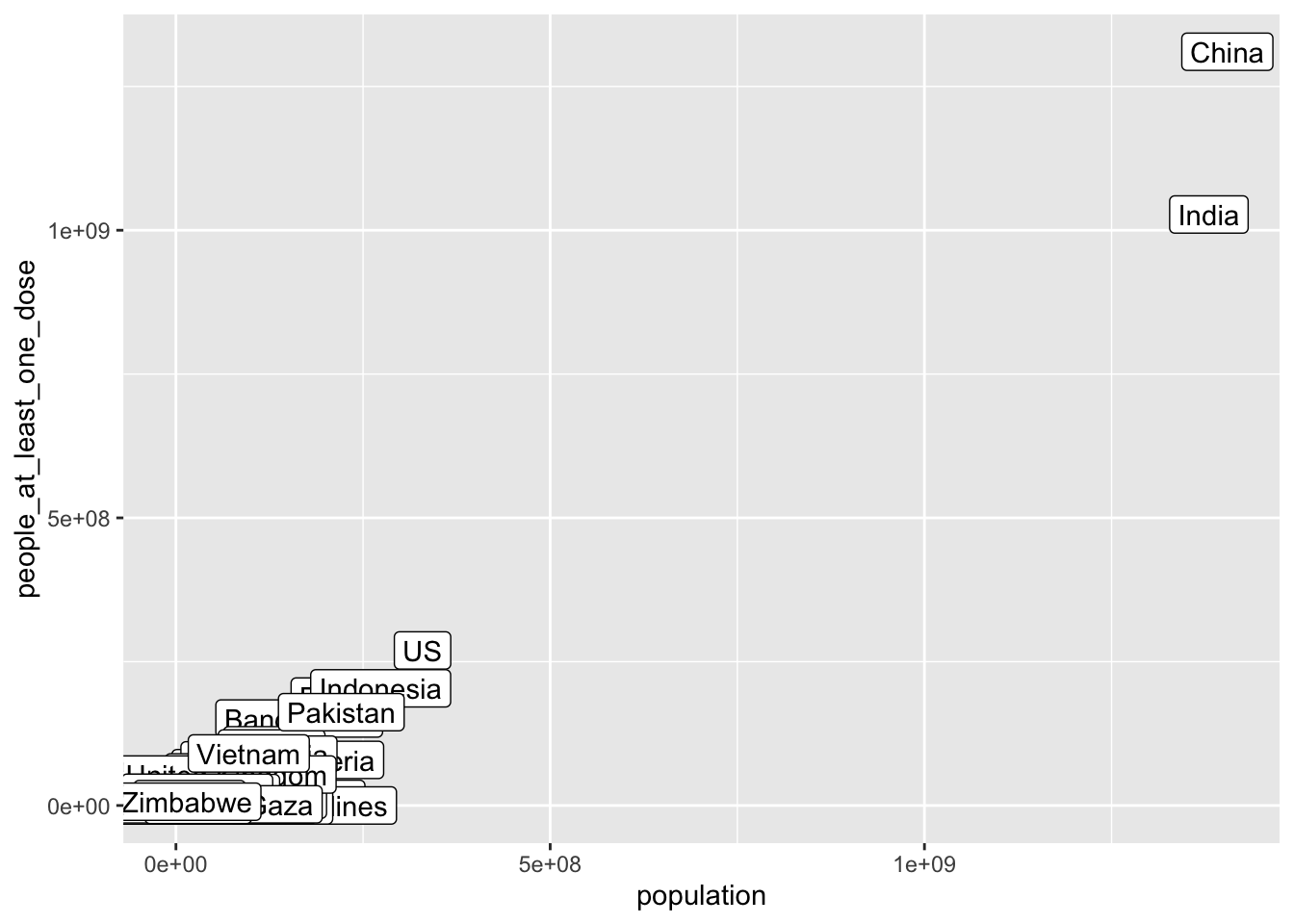## Exercise

Come up with an interesting question you want to explore in the Coronavirus dataset with a plot. Try to figure out how to plot it (remember: Google is your friend).

Examples of questions you could explore:

• We saw earlier that India has had a lower death count per number of confirmed cases than other countries, while Mexico had a higher death count per number of confirmed cases. Has this been a consistent pattern throughout the pandemic?

• For how long has the US been the country with the greatest number of confirmed cases?

• Have the global daily death counts peaked and declined or are they still rising? What about within individual countries?

## Some example plots:

#### Trend in cumulative case count

## linear scale
group_by(coronavirus, date, type) %>%
summarise(cases = sum(cases)) %>%
group_by(type) %>%
mutate(cases=cumsum(cases)) %>%
ggplot() +
geom_line(aes(x=date, y=cases, color=type))
## summarise() has grouped output by 'date'. You can override using the .groups argument.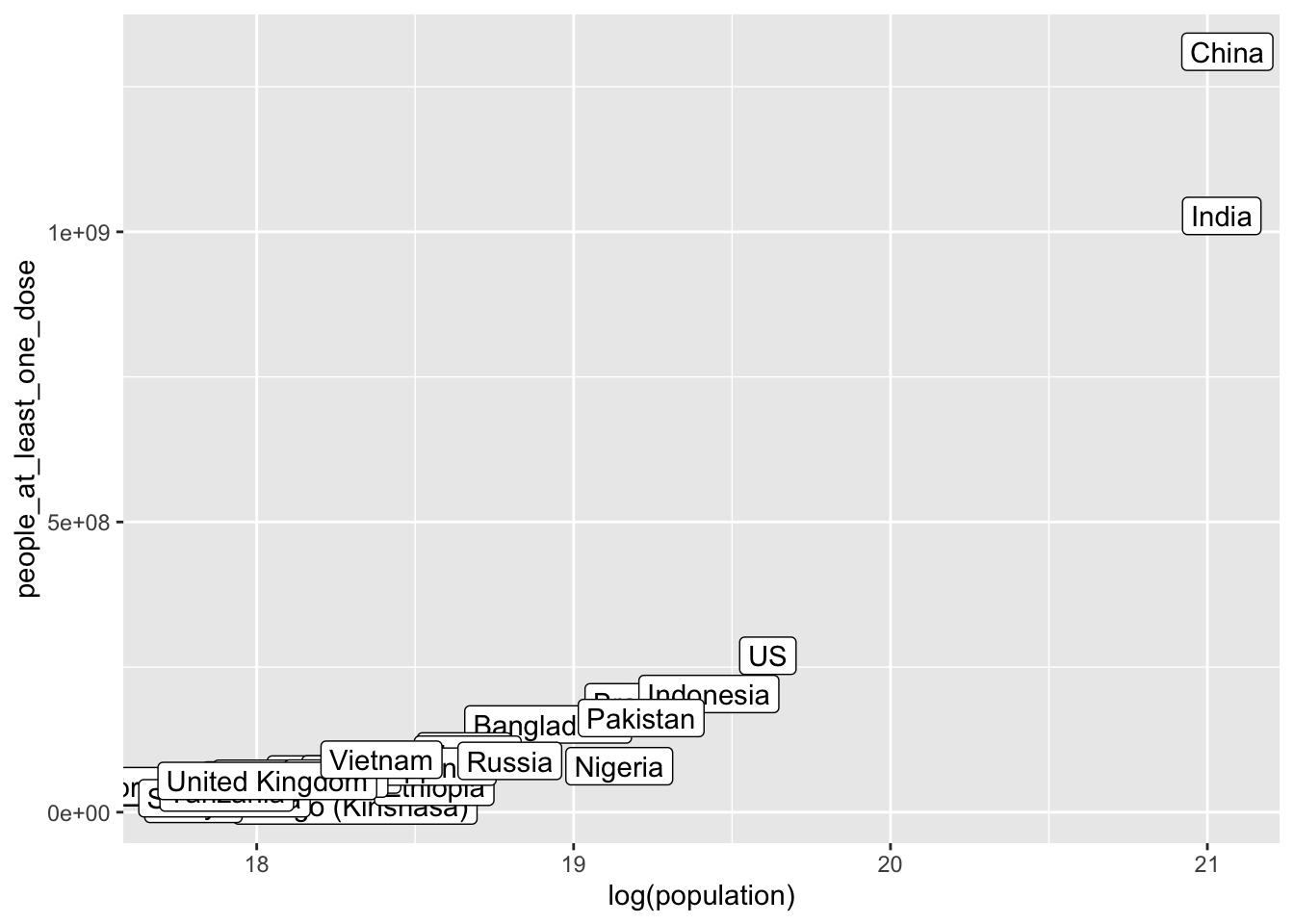## log scale
group_by(coronavirus, date, type) %>%
summarise(cases = sum(cases)) %>%
group_by(type) %>%
mutate(cases=cumsum(cases)) %>%
ggplot() +
geom_line(aes(x=date, y=log(cases), color=type))
## summarise() has grouped output by 'date'. You can override using the .groups argument.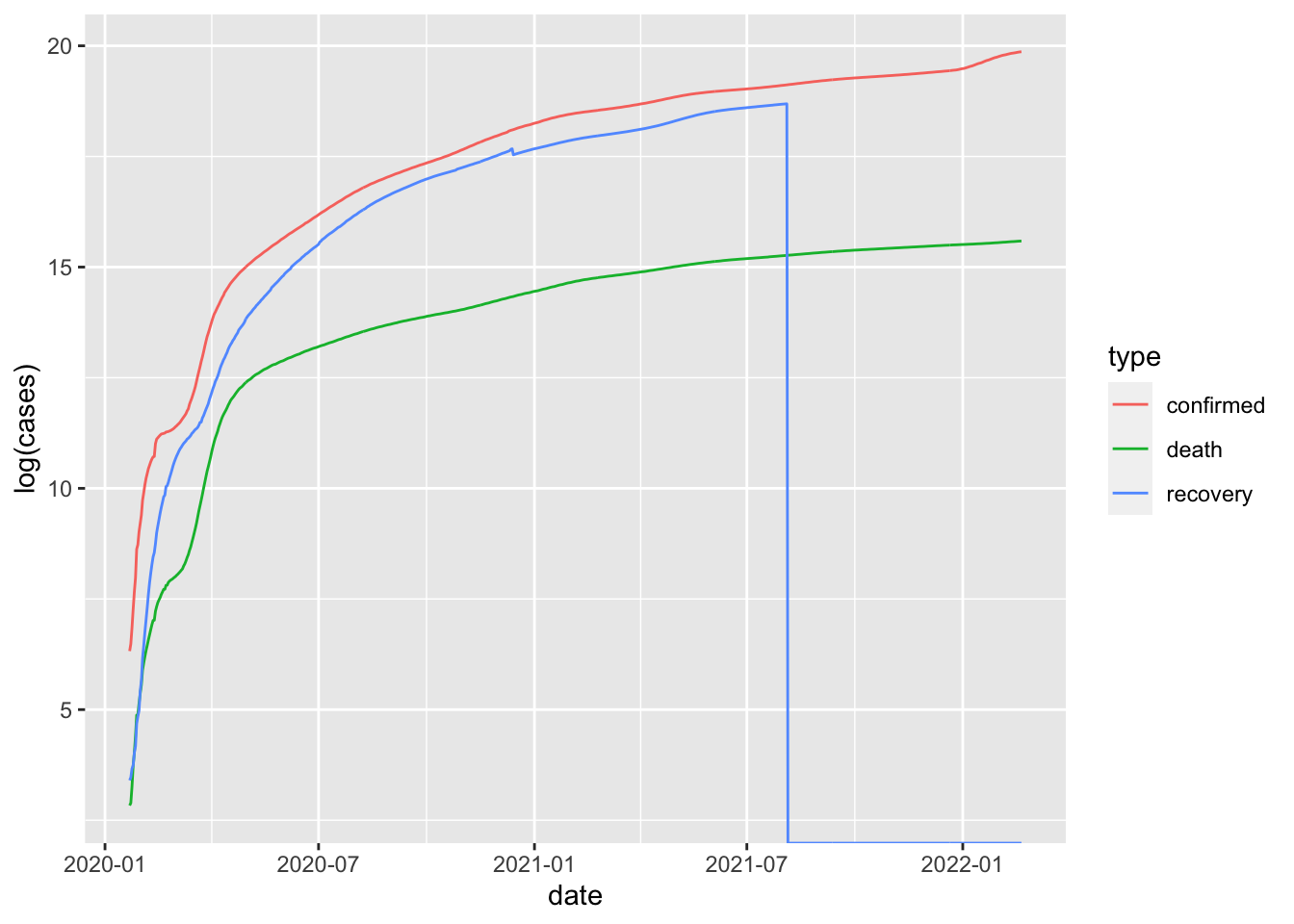#### Trend in cumulative case count in the 10 most infected countries

## linear scale
filter(coronavirus, country %in% top10_countries) %>%
group_by(country, date, type) %>%
summarise(cases = sum(cases)) %>%
group_by(country, type) %>%
mutate(cases=cumsum(cases)) %>%
ggplot() +
geom_line(aes(x=date, y=cases/1000, color=type)) +
ylab("cumulative count (in thousand)") +
facet_wrap(~country, scales = "free_y")
## summarise() has grouped output by 'country', 'date'. You can override using the .groups argument.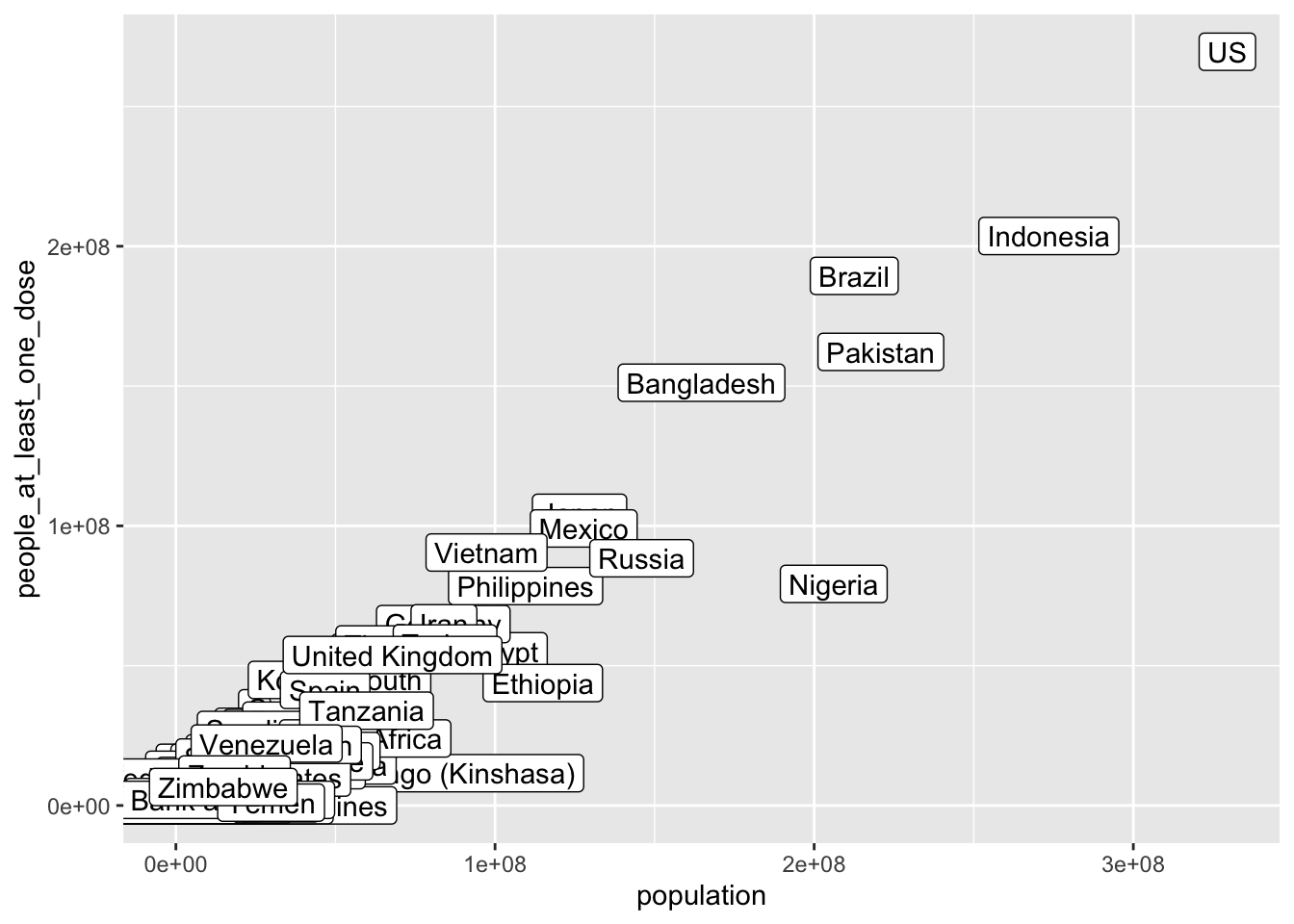#### Trend in cumulative death rate

group_by(coronavirus, date, type) %>%
summarise(cases = sum(cases)) %>%
group_by(type) %>%
mutate(cases=cumsum(cases)) %>%
pivot_wider(names_from = type, values_from = cases) %>%
mutate(death_rate = death/confirmed) %>%
ggplot(aes(x=date, y=death_rate)) +
geom_line()
## summarise() has grouped output by 'date'. You can override using the .groups argument.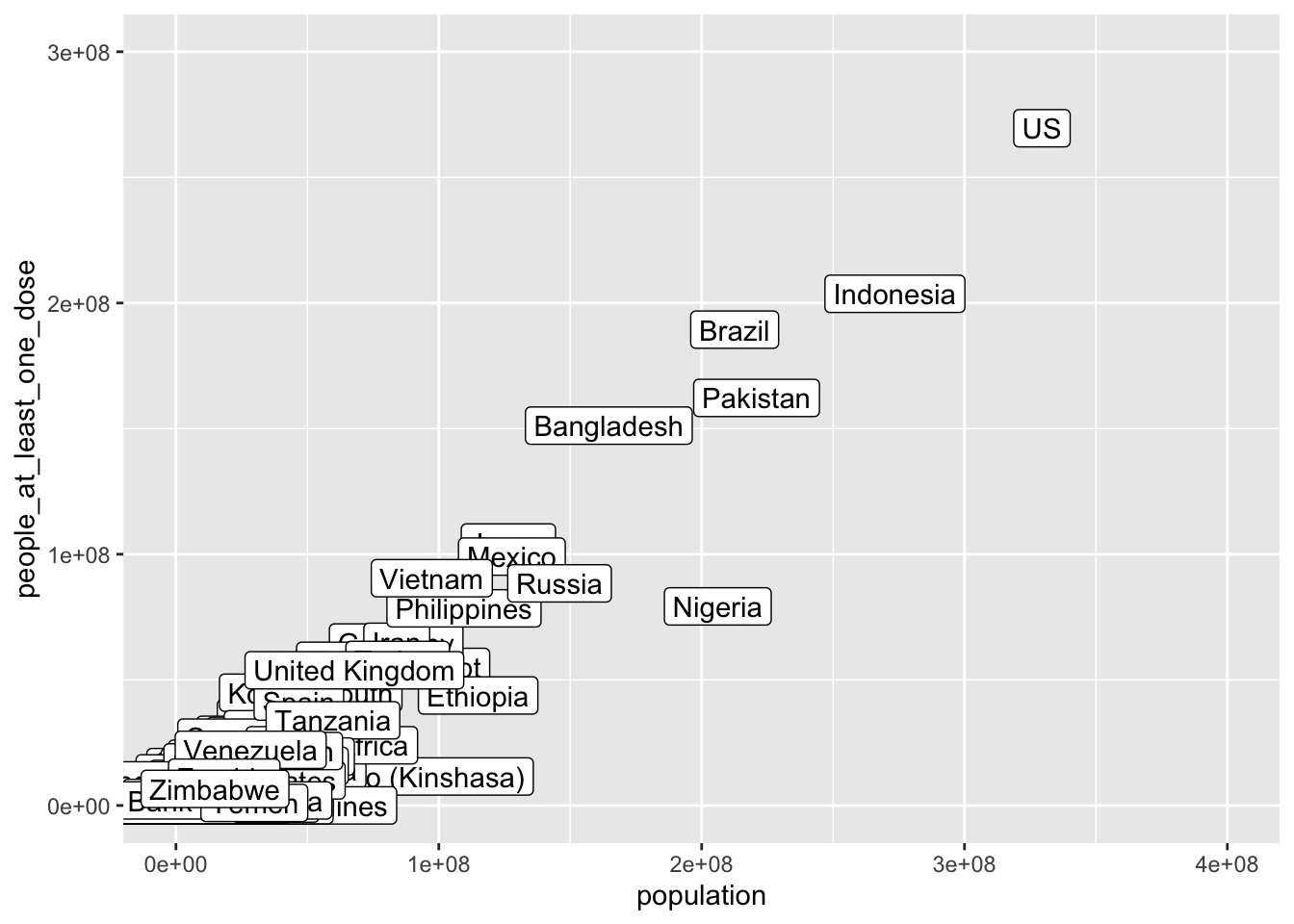#### Trend in cumulative death rate in 10 most infected countries

filter(coronavirus, country %in% top10_countries) %>%
group_by(country, date, type) %>%
summarise(cases = sum(cases)) %>%
group_by(country, type) %>%
mutate(cases=cumsum(cases)) %>%
pivot_wider(names_from = type, values_from = cases) %>%
mutate(death_rate = death/confirmed) %>%
ggplot(aes(x=date, y=death_rate)) +
geom_line() +
facet_wrap(~country, scales = "free_y")
## summarise() has grouped output by 'country', 'date'. You can override using the .groups argument.
## Warning: Removed 35 row(s) containing missing values (geom_path).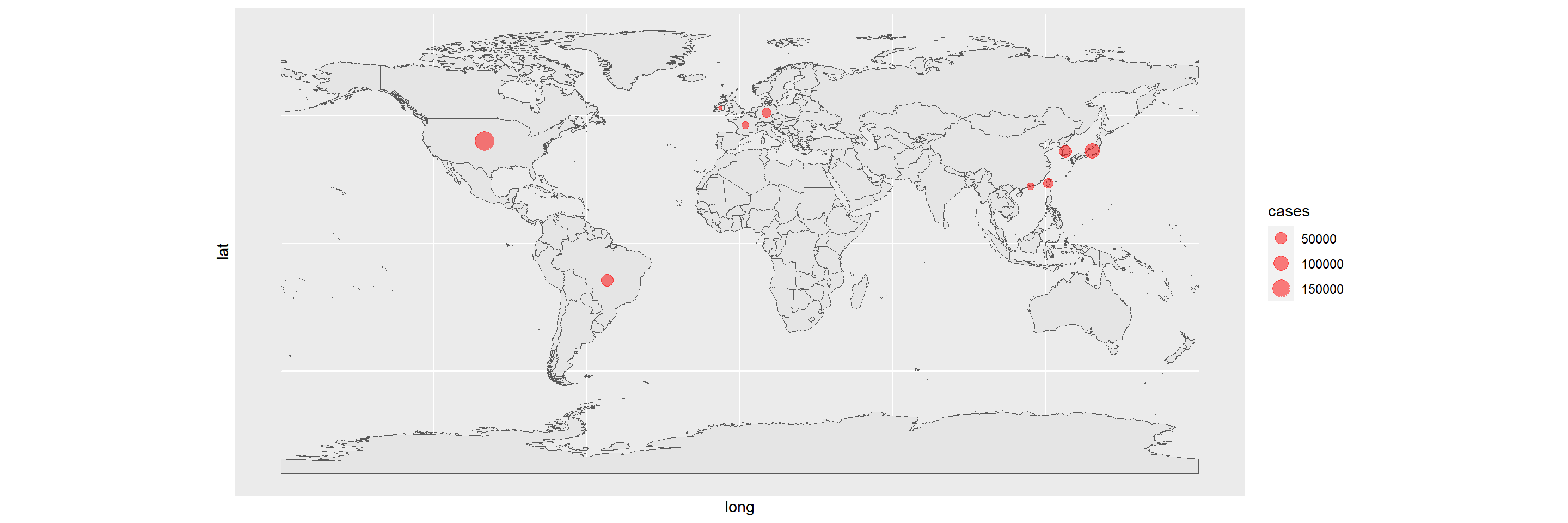#### Countries that had their first reported coronavirus case in January

filter(coronavirus, type=="confirmed", cases>0) %>%
group_by(country, date) %>%
summarise() %>%
group_by(country) %>%
filter(date==min(date)) %>%
ungroup() %>%
arrange(date) %>%
mutate(rank=row_number(date)) %>%
filter(months(date)=="January", lubridate::year(date)==2020) %>%
ggplot(aes(x=date, y=rank)) +
geom_label(aes(label=country)) +
theme_minimal()
## summarise() has grouped output by 'country'. You can override using the .groups argument.